# Introduction

This tutorial respects some writing conventions:

• menus, buttons, etc., are written in bold
(e.g. Algorithm / New Algorithm Window, OK, Definition list);
• command lines, SynDEx files, examples, etc., are written in Computer Modern
(e.g. -libs libs, examples/tutorial, ! int o);

To create an application workspace, launch SynDEx with option -libs libs. See the SynDEx v7 User Manual for more information.

The examples presented in this tutorial are located in the sub-folder examples/tutorial. Each example is located in its sub-folder. The example 7 is located in the examples/tutorial/example7, examples/tutorial/example7_mono, and examples/tutorial/example7_bi folders.

#### Example 1

• we create a sensor definition, an actuator definition, and a function definition. Then, we create an algorithm and define it as main. Finally, we create in the main algorithm two references to the sensor definition, three references to the actuator definition, and one reference to the function definition, and we create data dependences between these references by connecting their ports;
• we create four different architectures:
• an architecture with one operator,
• an architecture with two operators and a SAM point-to-point communication medium,
• an architecture with three operators and a SAM multipoint communication medium,
• an architecture with three operators and a RAM communication medium;
• we create constraints on the third architecture;
• we perform the adequation of the main algorithm onto the third architecture defined as main, without constraint and then with constraints.

#### Example 2

Hierarchy in algorithm:

• we create a function definition and a constant. Inside the function we create a reference to another function; in that way this definition is defined by hierarchy. Then, we create a third function that references both previous ones. Finally, we create an algorithm that references the third function and define it as main. In that way, the main algorithm references a hierarchical function;
• we create parameters names for an operation, and assign values to these parameters.

#### Example 3

Delay in algorithm:

• we create a delay definition;
• we create a main algorithm by referencing a delay, a sensor, an actuator, and a function and by connecting them.

#### Example 4

Repetition and library in algorithm:

• we create a multiplication function of a vector by a scalar by repeating a multiplication function on scalars:
• firstly without any library,
• secondly with a library;
• we create a multiplication function of a matrix by a vector by repeating a multiplication function on vectors.

#### Example 5

Condition and nested condition in algorithm:

• we create an algorithm conditioned by a data dependence, the value of which indicates the operation to be executed;
• we create an algorithm conditioned by a data dependence, one operation of which is in turn conditioned (nested condition) by the same data dependence.

#### Example 6

Algorithm, architecture, adequation, and code generation:

• we create a main algorithm by referencing a sensor, two actuators, three functions, and a constant and by connecting them;
• we create an architecture with two operators of type U and a communication medium of type u/TCP;
• we perform the code generation, then we create manualy the example6.m4x file (for operations not defined in libraries);
• we create manualy the example6.m4m file (to define the hostname) and the root.m4x file (for the main operator);
• we create manualy the GNUmakefile, then we execute the executives created after compilation.

#### Example 7

Definition of the source code into the code editor window:

• we add parameters to the conv function of Example 6;
• we modify the code associated with this function first in case of a generic processor then in case of an architecture with heterogenous processors;
• we create manualy the example7.m4m file (to define the hostname) and the root.m4x file (for the main operator);
• we create manualy the GNUmakefile;
• we perform the adequation, then we perform compilation, finally we compile the executives and launch the executables.

Definition of the source code in separate C files:

• we define the code of a new function in a .c file,
• we define the code of a new function in a .c file and we use a .h file.

#### Example 8

A complete realistic application from adequation to execution:

• we build the model of a complete application for two cars;
• we generate the code for each processor;
• we compile and execute the code associated with each processor.

#### Example 9

A multiperiodic application:

• we build a basic multi-periodic application
• mono-phase schedule
• multi-phase schedule

# Chapter 1  Example 1: algorithm, architecture, and adequation

## 1.1  The main algorithm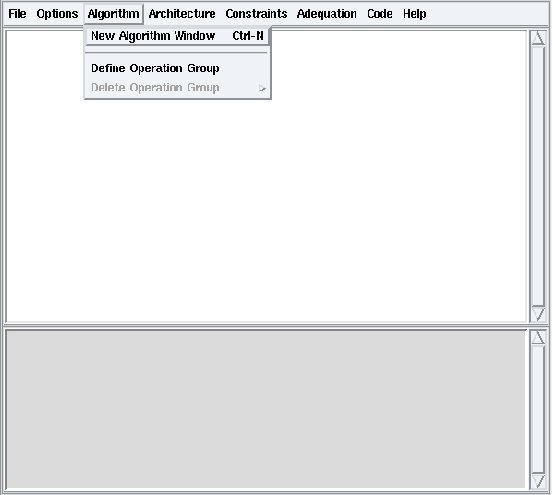Figure 1.1: Algorithm / New Algorithm Window

From the principal window, choose the File / Save as option and save your first application under a new folder (e.g. my_tutorial) with the name example1.

Choose Algorithm / New Algorithm Window (cf. figure 1.1). It opens the edition window for algorithm definitions.

### 1.1.1  Definition of a sensor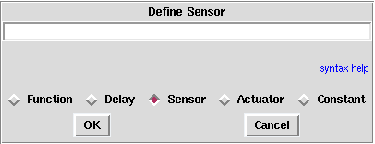Figure 1.2: Define Sensor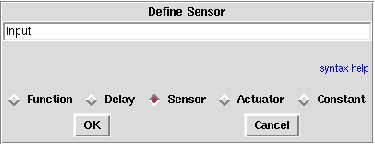Figure 1.3: Name of the new sensor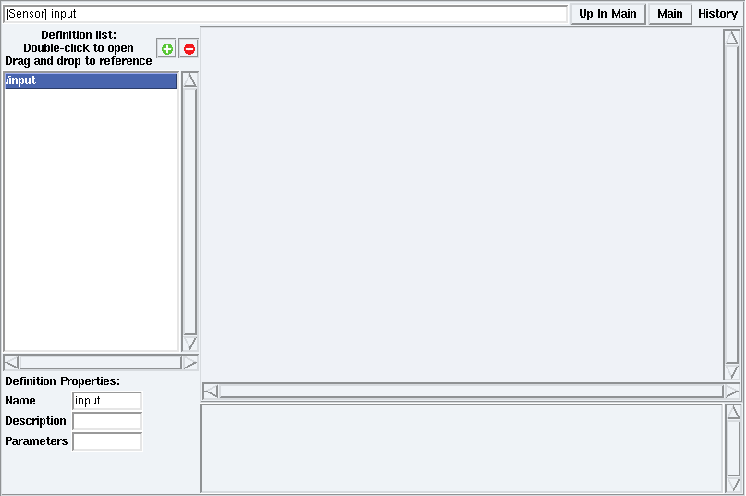Figure 1.4: Sensor definition window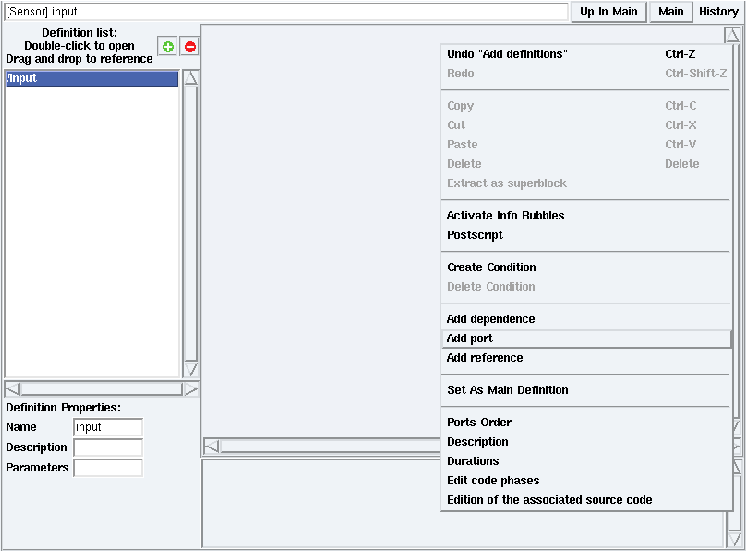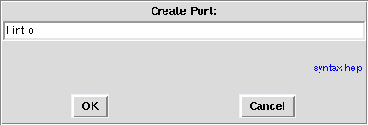Figure 1.6: Create Port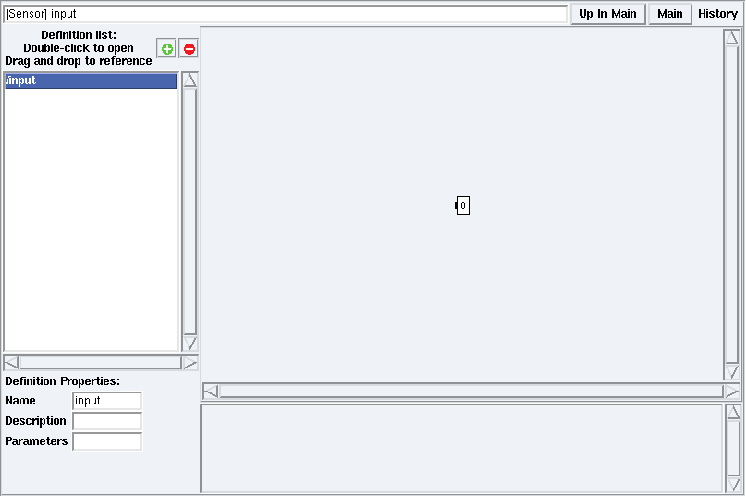Figure 1.7: Sensor definition window after output port created

To create an input sensor definition:

• from the algorithm window, click on the + green button. It opens a dialog window, check Sensor (cf. figure 1.2). Type the sensor name and optionally a list of parameters for the sensor. For example type input, then click OK (cf. figure 1.3). It creates the definition of the input sensor. To open it in definition mode, double click on input in the Definition list (cf. figure 1.4);
• in input definition mode, right click on the background and select Add port (cf. figure 1.5). It opens a dialog window for the port’s direction, type, name and optionally its size. For example type ! int o, then click OK (cf. figure 1.6). It creates the integer output port o (cf. figure 1.7) in the sensor definition window.

### 1.1.2  Definition of an actuator

To create an output actuator definition:

• from the algorithm window, click on the + green button → dialog window: check Actuator then type output and click OK;
• double click on output in the Definition list. Then right click on its background and select Add port → dialog window: ? int i. Click OK. It creates the integer input port i in the sensor definition window.

### 1.1.3  Definition of a function

To create a computation function definition:

• from the algorithm window, click on the + green button → dialog window: check Function then type computation and click OK;
• double click on computation in the Definition list. Thenright click on its background and select Add port → dialog window: ? int a ? int b ! int o. Click OK. It creates the integer ports a, b, and o in the function definition window.

### 1.1.4  Definition of the main algorithm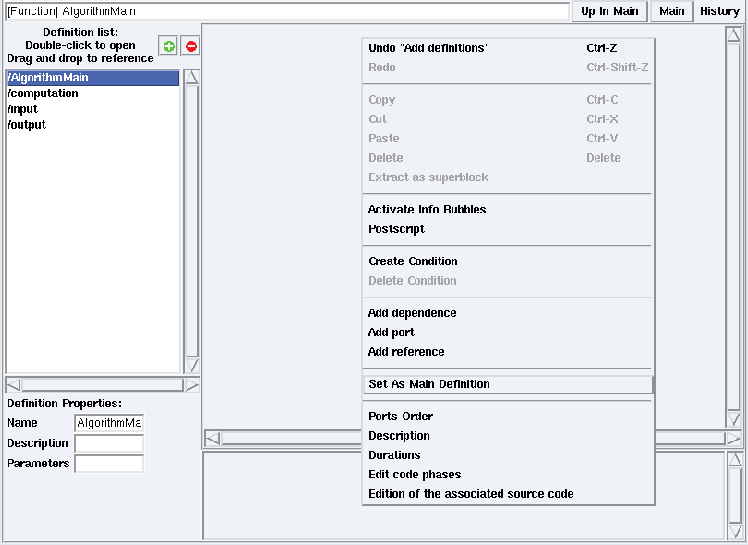Figure 1.8: Contextual menu → Set As Main Definition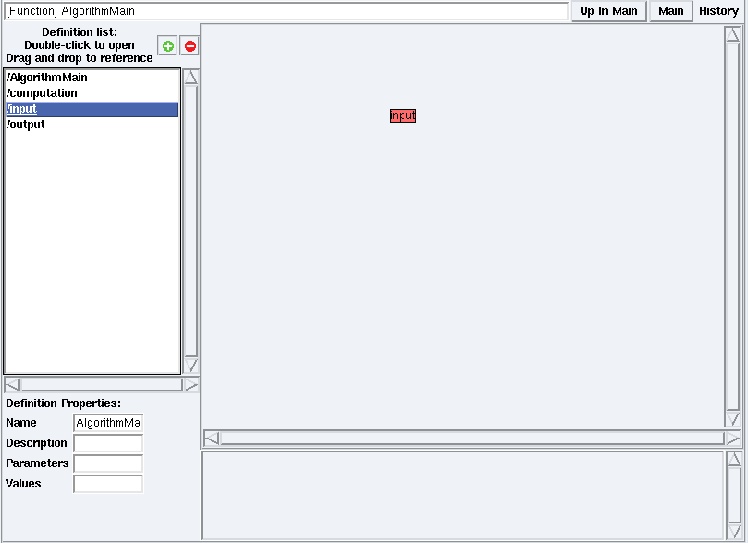Figure 1.9: Drag and drop input definition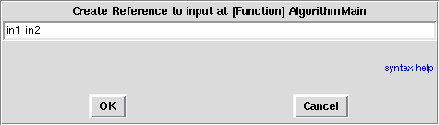Figure 1.10: Create References to inputFigure 1.11: Main algorithm after references to sensor created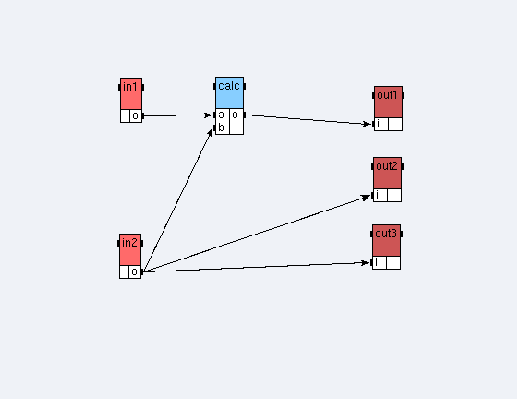Figure 1.12: Main algorithm of the Example 1

To create an AlgorithmMain function definition:

• from the algorithm window, click on the + green button → dialog window: check Function then type AlgorithmMain and click OK;
• double click on AlgorithmMain in the Definition list. Then right click on its background and select Set As Main Definition (cf. figure 1.8);
• in its definition mode,
• to create references to the sensor input, drag and drop the sensor definition from the Definition list to the AlgorithmMain definition window (cf. figure 1.9) → dialog window: in1 in2 (cf. figure 1.10). The main algorithm looks like the figure 1.11,
• to create references to the actuator output, drag and drop the actuator definition from the Definition list to the AlgorithmMain definition window → dialog window: out1 out2 out3,
• to create a reference to the function computation, drag and drop its definition → dialog window: calc,
• from the AlgorithmMain definition window, to create a data dependence between in1 and calc, point the cursor on the output port o of the in1 operation, middle click, and drag to the input port a of the calc operation. It draws an arrow between these target ports. After creating the other data dependences, the main algorithm looks like the figure 1.12.

## 1.2  An architecture with one operator

### 1.2.1  Definition of an operator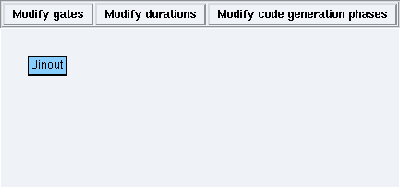Figure 1.13: Operator definition window

To create an Uinout operator definition:

• from the principal window, choose Architecture / Define Operator. It opens a dialog window, type Uinout and click OK. It opens the operator definition window (cf. figure 1.13);
• from the Uinout definition window:
• to add a gate: click Modify gates → dialog window: gate_type_1 x,
• to set the operator execution durations: click Modify durations → dialog window:
```
computation = 2
input = 1
output = 3

```

### 1.2.2  Definition of the main architecture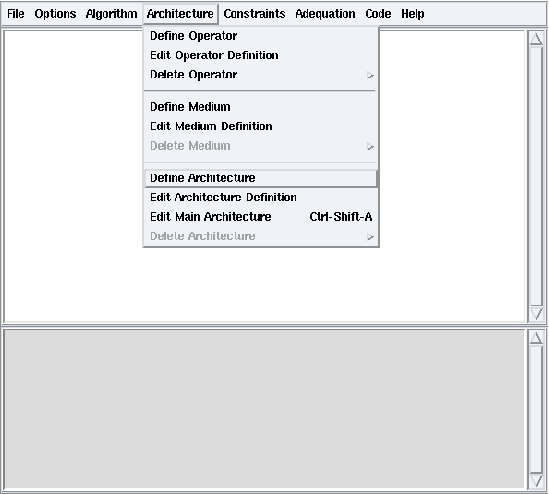Figure 1.14: Architecture / Define Architecture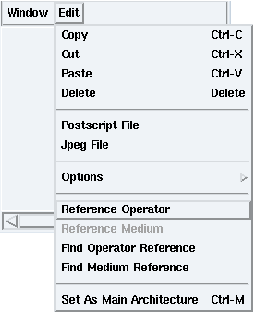Figure 1.15: Edit / Reference Operator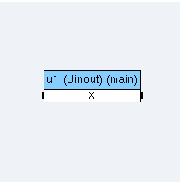Figure 1.16: Architecture with one operator

To create an ArchiOneOperator architecture definition:

• from the principal window: Architecture / Define Architecture (cf. figure 1.14) → dialog window: type ArchiOneOperator then click OK → definition window;
• from the ArchiOneOperator definition window:
• to create a reference to the operator Uinout, Edit / Reference Operator (cf. figure 1.15) → dialog window: click user, double click Uinout → dialog window: u1,
• to define the operator as main, right click on its reference and select Set As Main Operator.

The architecture looks like the figure 1.16.

## 1.3  An architecture with a SAM point-to-point comunication medium

### 1.3.1  Definition of operators

To create Uin and Uout definitions:

• from the principal window: Architecture / Define Operator → dialog window: Uin, click OK → definition window;
• from the Uin definition window:
• click Modify gates → dialog window:
```
MediumSamPointToPoint x
MediumSamMultiPoint y
MediumRam z

```
• click Modify durations → dialog window:
```
computation = 2
input = 2
output = 5

```
• from the principal window: Architecture / Define Operator → dialog window: Uout, click OK → definition window;
• from the Uout definition window:
• click Modify gates → dialog window:
```
MediumSamPointToPoint x
MediumSamMultiPoint y
MediumRam z

```
• click Modify durations → dialog window:
```
computation = 2
input = 5
output = 3

```

### 1.3.2  Definition of a medium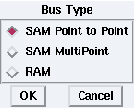Figure 1.17: Type of a communication medium

To create a MediumSamPointToPoint medium definition:

• from the principal window: Architecture / Define Medium → dialog window: MediumSamPointToPoint, click OK → definition window;
• from the MediumSamPointToPoint definition window:
• click Modify type → dialog window: SAM Point to Point (cf. figure 1.17),
• click Modify durations → dialog window:
```
float = 2
int = 2
uchar = 1
ushort = 1

```

### 1.3.3  Definition of the main architecture

To create an ArchiSamPointToPoint architecture definition:

• from the principal window: Architecture / Define Architecture (cf. figure 1.14) → dialog window
ArchiSamPointToPoint → definition window;
• from the ArchiSamPointToPoint definition window, create references u1 and u2 to the operators Uin and Uout;
• from the ArchiSamPointToPoint definition window: Edit / Reference Medium → dialog window: click user, select MediumSamPointToPoint → dialog window: type medium_sampp;
• define the operator u1 as main.

### 1.3.4  Connections between the operators and the medium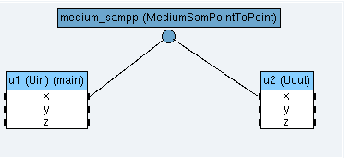Figure 1.18: Architecture with two operators and a SAM point-to-point communication medium

In the main architecture window, to create a connection between the u1 operator and the medium_sampp medium, point the cursor on the port x of the operator, middle click, and drag it to the communication medium. It draws an edge between the operator and the communication medium. After creating the other connection, the main architecture looks like the figure 1.18.

## 1.4  An architecture with a SAM multipoint medium

To create an ArchiSamMultiPoint architecture definition:

• from the principal window: Architecture / Define Architecture (cf. figure 1.14) → dialog window: ArchiSamMultiPointArchiSamMultiPoint definition window;
• create references u1 and u2 to the operator Uin and a reference u3 to the operator Uout, like in the previous example;
• create a medium definition MediumSamMultiPoint of type SAM MultiPoint with durations:
```
float=2
int=2
uchar=1
ushort=1

```
• create a reference medium_sammp to this medium in the main architecture window → dialog window: check No Broadcast;
• define the operator u1 as main;
• connect the operators ports y to the medium.

The architecture looks like the figure 1.19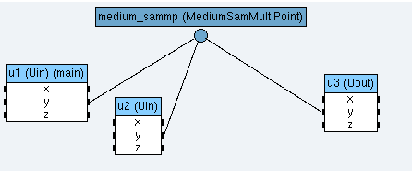Figure 1.19: Architecture with three operators and a SAM multipoint communication medium

## 1.5  An architecture with a RAM medium

To create the ArchiRam architecture definition:

• from the principal window: Architecture / Define Architecture (cf. figure 1.14) → dialog window ArchiRamArchiRam definition window;
• create a reference u1 to the operator Uin and references u2 and u3 to the operator Uout;
• create a medium definition MediumRam of type RAM with durations
```
float=2
int=2
uchar=1
ushort=1

```
and create a reference medium_ram in the main architecture;
• define the operator u1 as main;
• connect the operators ports z to the medium.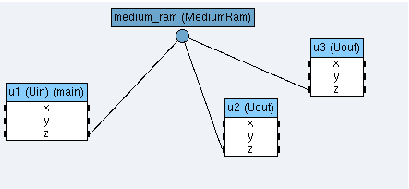Figure 1.20: Architecture with three operators and a RAM comunication medium

The architecture looks like the figure 1.20.

### 1.6.1  Without constraint

Define the architecture with three operators and a medium of type SAM MultiPoint (cf. 1.4) as main architecture (Edit / Set As Main Architecture).

It opens the schedule window (cf. figure 1.21) in which you can see the schedule of the algorithm on the architecture and the schedule of the different inter-operator communications on the medium.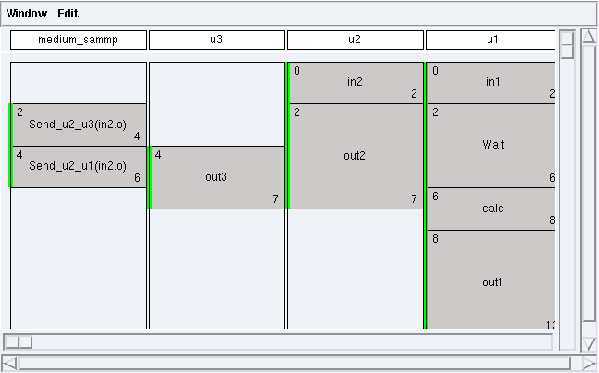Figure 1.21: Schedule

### 1.6.2  With constraints

To contraint the ArchiSamMultiPoint architecture:

• from the principal window, to create the constraints:
• Algorithm / Define Operation Group (cf. figure 1.22) → dialog window: og1 og2 og3,
• Constraints / Absolute Constraints → dialog window: select ArchiSamMultiPoint It opens a dialog window in which you can create constraints on the different operators of the architecture selected:
• first click on og1, then u1, and the Create button, to constrain the operation group og1 on the operator u1,
• constrain the operation group og2 on the operator u2,
• constrain the operation group og3 on the operator u3,
• click on OK button (cf. figure 1.23),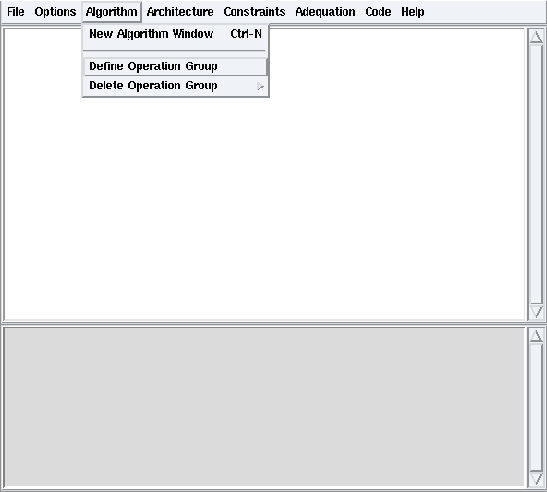Figure 1.22: Define Operation Group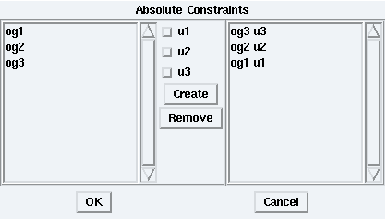Figure 1.23: Constrain operation groups on operators of the architecture selected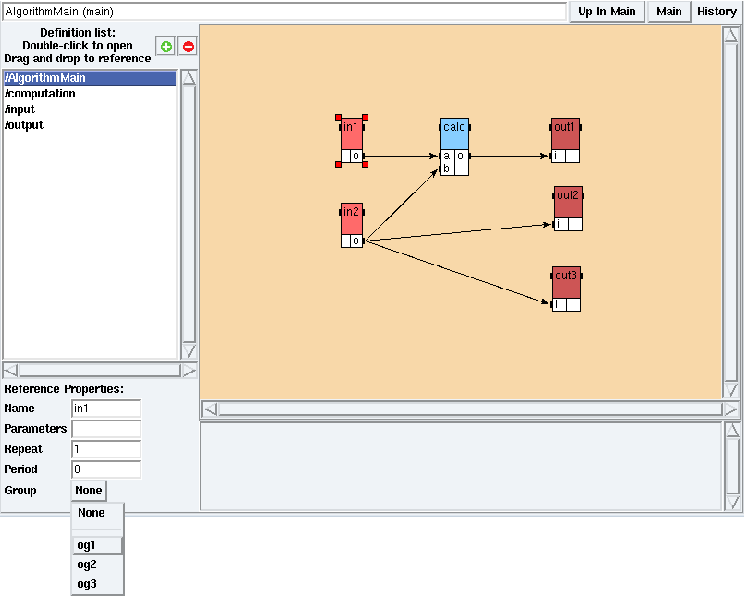Figure 1.24: Attach a reference to an operation group

• in the main mode (Main button):
• select the operation in1, click on the Group button of its Reference Properties then select og1 (cf. figure 1.24),
• attach the operation in2 to the operation group og2,
• attach the operation out1 to the operation group og1,
• attach the operation out2 to the operation group og2,
• attach the operation out3 to the operation group og3,
• attach the operation calc to the operation group og3;

The algorithm with constraints looks like the figure 1.25.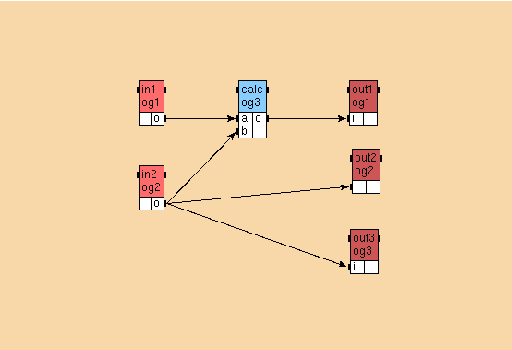Figure 1.25: Algorithm with constraints

• from the principal window, to perform the adequation with constraints: Adequation / Launch Adequation, then Adequation / Display Schedule → schedule window. The schedule looks like the figure 1.26.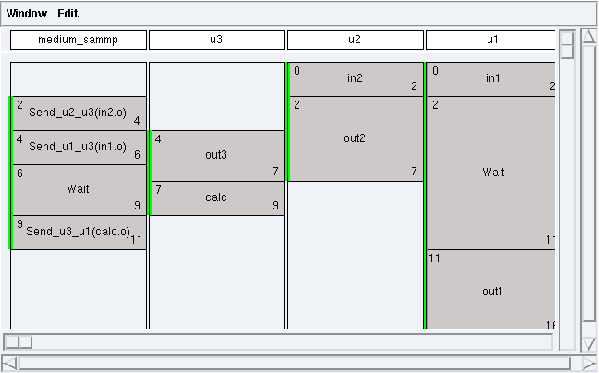Figure 1.26: Schedule with constraints

From the principal window, choose File / Close. In the dialog window, click on the Save button.

# Chapter 2  Example 2: parameters and hierarchy in algorithm

From the principal window, choose File / Save as and save your second application under your tutorial folder with the name example2.

## 2.1  Definition of the function A

To create the A function definition:

• from the algorithm window:
• click on the + green button → dialog window: check Function then type A and click OK,
• click on the + green button → dialog window: check Constant then type constante<X> and click OK. Create an integer output port o inside;
• in the A definition window:
• create a reference cst<T> to the definition constante,
• create an integer input port a, an integer output port o (Contextual menu → Add port cf. 1.1.3);
• from the algorithm window,
create a function definition calcul1, with two integer input ports a and b and an integer output port o;
• in the A definition window:
• create a reference calc1 to the definition calcul1,
• add a parameter name (cf. figure 2.1): Field ParametersT.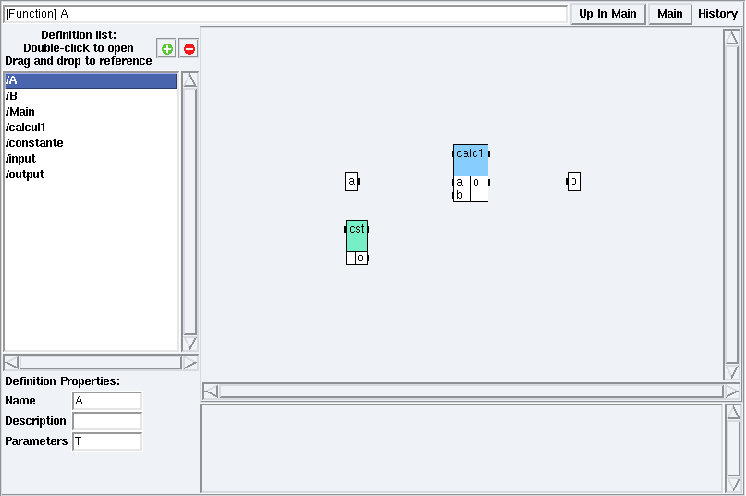Figure 2.1: Parameters

The function A looks like the figure 2.2.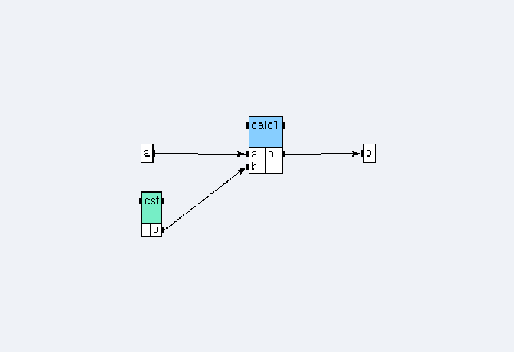Figure 2.2: Function A

## 2.2  Definition of the function B

To create the B function definition:

• from the algorithm window: + green button → dialog window: B<X;Y>;
• in the B definition window:
• create references A1 and A2 to the definition A (A1<X> A2<Y>),
• create two integer input ports a and b, one integer output port o, and the function calc1 of the definition calcul1,
• add the parameters names X and Y (field Parameters).

The function B looks like the figure 2.3.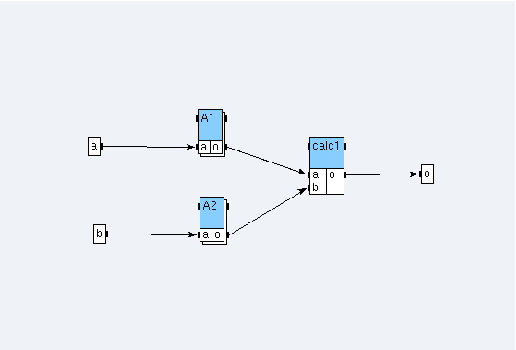Figure 2.3: Function B

## 2.3  Definition of the algorithm with hierarchy

To create the Main algorithm:

• from the algorithm window: + green button → dialog window: Main;
• from the definition window, define it as main;
• create a sensor input with an integer output port o and an actuator output with an integer input port i;
• in the Main algorithm window, create a reference B<1;2> to the definition B, a reference i1 i2 to the definition input, and a reference out to the definition output;
• create dependences between the references.

The algorithm looks like the figure 2.4.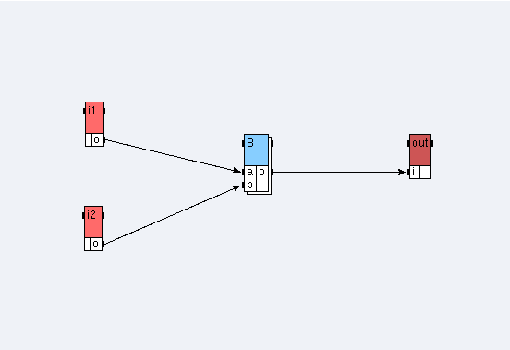Figure 2.4: Algorithm of the Example 2

From the principal window, choose File / Close. In the dialog window, click on the Save button.

# Chapter 3  Example 3: delay in algorithm

From the principal window, choose File / Save as and save your third application under your tutorial folder with the name example3.

## 3.1  Definition of the operations input, output, and calc

Create a sensor input, an actuator output, and the function calc, like in the Examples 1 and 2. (cf. 1.1.3 and calcul1 in 2.1)

## 3.2  Definition of the delay

To create the calcPrec delay:

• from the algorithm window, click on the + green button → dialog window: check Delay then type calcPrec<init;size> and click OK; The parameter init will be used to specify the initial value of the delay, and size the number of delays;
• in the definition window, create one input port and one output port. Enter: ? int i ! int o.

## 3.3  Definition of the algorithm with delay

Create an algorithm algorithmMain. Create a reference in1 to the definition input, a reference calc to the definition calc, a reference out1 to the definition output, and a reference calcPrec<0;1> to the definition calcPrec. Create dependences between the references.

The algorithm looks like the figure 3.1.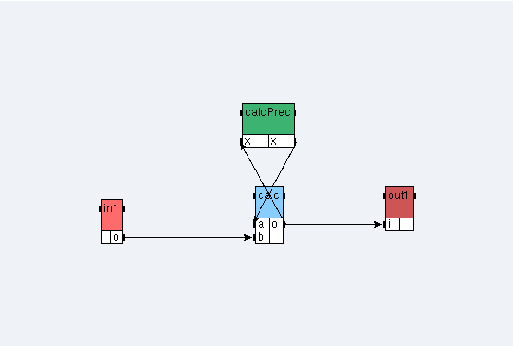Figure 3.1: Algorithm of the Example 3

From the principal window, choose File / Close. In the dialog window, click on the Save button.

# Chapter 4  Example 4: repetition and library in algorithm

From the principal window, choose File / Save as and save your fourth application under your tutorial folder with the name example4.

## 4.1  An algorithm with repetition without any library

In this section, we create a multiplication function of a N elements vector by a scalar by repeating N times a multiplication function on scalars.

### 4.1.1  Definition of the scalar ins and the function mul on scalars

In a new algorithm window:

• create a new sensor definition named ins with an integer output port o;
• create a new function definition named mul with two integer input ports a and b and an integer ouput port o).

### 4.1.2  Definition of the vectors inv and outv

To create the vectors:

• to create the definition inv:
• from the algorithm window, create a new sensor definition named inv,
• from the inv definition window, type N in the Parameters textfield of its Definition Properties (it has N elements),
• create its integer output port named o with length N: ! int[N] o;
• to create the definition outv:
• from the algorithm window, create a new actuator definition outv,
• in outv definition window, type N in the Parameters textfield of its Definition Properties (it has N elements),
• create its integer input port named i with length N: ? int[N] i.

### 4.1.3  Definition of the algorithm AlgorithmMain1

To create the AlgorithmMain1 algorithm:

• from the algorithm window, create a new function definition named AlgorithmMain1 and from its definition window, define it as main;
• in the AlgorithmMain1 definition mode:
• create a reference s_input to the scalar ins,
• create a reference v_input<N> to the vector inv,
• create a reference mul to the function mul and type N in the Repeat textfield of its Reference Properties (it is repeated N times),
• create a reference v_output<N> to the vector outv;
• create dependences between the references, in order to obtain the main algorithm (cf. figure 4.2);
• type N in the Parameters textfield of the Definition Properties of the main algorithm and 3 in the Values textfield (cf. figure 4.1). Notice that this value is keeped as long as the algorithm remains the main one.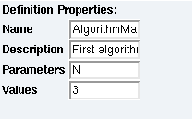Figure 4.1: Parameters Values

The repetition consists in multiplying each of the 3 elements of the v_input vector with the s_input scalar and placing the result in the 3 elements v_output vector.

The parameter N is here the repetition factor of the mul function.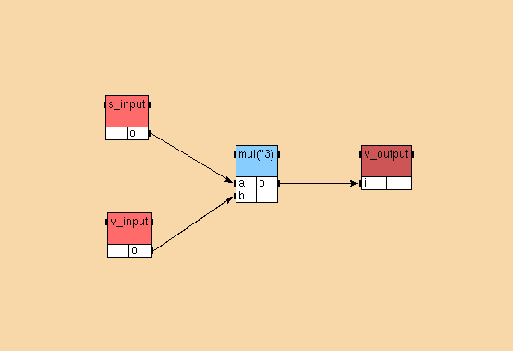Figure 4.2: AlgorithmMain1 of the Example 4

## 4.2  An algorithm with repetition with the int library

In this section, we create a multiplication function of a vector by a scalar by using the int library.

### 4.2.1  Inclusion of the library int

From the principal window choose File / Specify Library Directories and add the SYNDEXPATH/libs where SYNDEXPATH is the absolute path of the SynDEx distribution.

From the principal window, choose File / Included Libraries / int (cf. figure 4.3).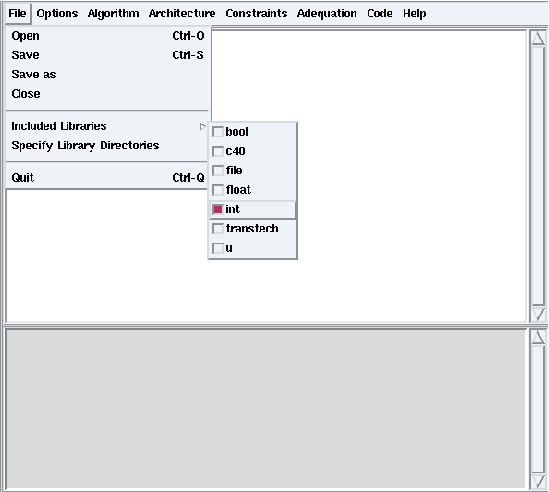Figure 4.3: File / Included Libraries / int

### 4.2.2  Definition of the algorithm AlgorithmMain2

Notice that this library contains input, mul, and output definitions parameterized with length.

We will need to set it to 1 for the scalar and the multiplication function, and to N for the vectors:

• from the algorithm window, create the function definition AlgorithmMain2 and define it as main;
• in AlgorithmMain2 definition mode:
• drag and drop the sensor definition int/input from the Definition list to the AlgorithmMain2 window → dialog window: s_input<1> (cf. figure 4.4) (it is a scalar),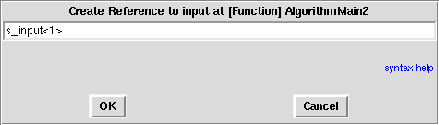Figure 4.4: Create Reference to int/input

• drag and drop the sensor definition int/input → dialog window: v_input<N> (it has N elements),
• drag and drop the function definition int/Arit_mul → dialog window: mul<1> then type N in the Repeat textfield of its Reference Properties, (it is a multiplication on scalars, repeated N times),
• drag and drop the actuator definition int/output → dialog window: v_output<N> (it has N elements);
• create dependences between the references in order to obtain the main algorithm (cf. figure 4.5);
• type N in the Parameters textfield of the Definition Properties, and 3 in the Values textfield.

Notice the difference of the mul reference when it is seen from the AlgorithmMain2 definition mode or from the main mode (Main button).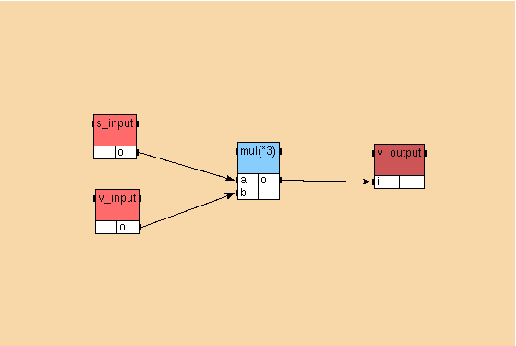Figure 4.5: AlgorithmMain2 of the Example 4

## 4.3  An algorithm with repetition with the float library

In this section, we create a multiplication function of a N*M matrix by a M elements vector by repeating N times a multiplication function on vectors.

### 4.3.1  Inclusion of the library float

Include the library float (File / Included Libraries / Float).

### 4.3.2  Definition of the function dpacc

This function is a multiplication function on scalars with an accumulator:

• create a new function definition named dpacc;
• create a reference mul<1> to the function float/Arit_mul (the reference works on scalars);
• create a reference add<1> to the function float/Arit_add (the reference works on scalars);
• add it three input ports and one output port: ? float s1 ? float s2 ? float acc ! float acc;
• then create dependences to obtain an algorithm (cf. figure 4.6).

Notice that acc is an input port and an output port of the function. It will be used as an accumulator to store the partial sum.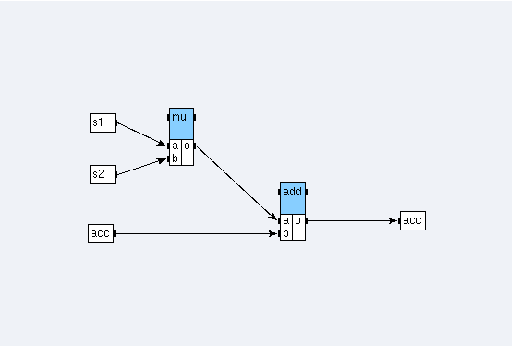Figure 4.6: Algorithm of the function dpacc

### 4.3.3  Definition of the function dp

This function is a multiplication function on vectors with an accumulator:

• create a new function definition named dp;
• add it a parameter dpaccn;
• create a reference zero<{0}> to the constant float/cst (it is the {0} scalar);
• create a reference dpacc to the function dpacc then type dpaccn in the Repeat textfield of its Reference Properties (it is repeated dpaccn times);
• add it two input ports: ? float[dpaccn] v1 ? float[dpaccn] v2 and one output port: ! float dp (vectors have dpaccn elements);
• create dependences to obtain an algorithm (cf. figure 4.7). To build the dependence between the output port acc of dpacc and its input port acc, choose Iterate on the dialog window (it is the connection between two successive calls of the function).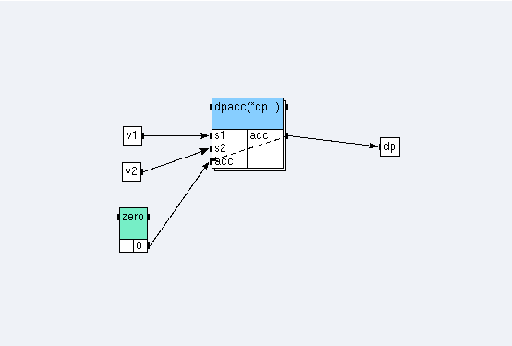Figure 4.7: Algorithm of the function dp

The repetition consists in multiplying two dpaccn elements vectors by calling dpaccn times the dpacc multiplication function on scalars with accumulator. The initial value of its accumulator is given by the zero constant and the following are given by the accumulator itself.

### 4.3.4  Definition of the function prodmatvec

This function is a multiplication function of a matrix by a vector:

• create a new function definition named prodmatvec;
• type a;b in the Parameters textfield of its Definition Properties;
• create a reference dotprod<b> to the function dp (input vectors have b elements) and type a in the Repeat textfield of its Reference Properties (it is repeated a times);
• add it two input ports: ? float[a*b] inm ? float[b] inv and one output port: ! float[a] outv;
• then create dependences to obtain an algorithm (cf. figure 4.8).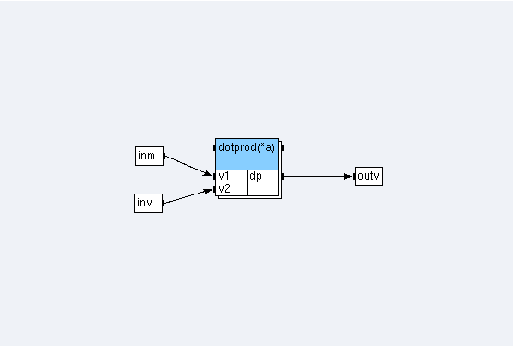Figure 4.8: Algorithm of the function prodmatvec

The repetition consists in multiplying a a*b matrix by a b elements vector by calling a times the dp multiplication function on vectors.

### 4.3.5  Definition of the algorithm AlgorithmMain3

To create the AlgorithmMain3 algorithm:

• create the definition of the sensor inm, with two parameters names N and M, and with an output port: ! float [N*M] o;
• create a new function definition named AlgorithmMain3 and define it as main;
• add it two parameters:N;M with values 3;4;
• create a reference m1<N;M> to the matrix inm;
• create a reference inv<N> to the vector float/input;
• create a reference matprodvec<N;M> to the function prodmatvec;
• create a reference outv<M> to the vector float/output;
• then create dependences to obtain an algorithm (cf. figure 4.9).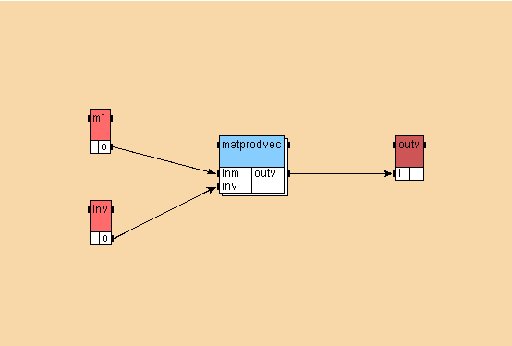Figure 4.9: AlgorithmMain3 of the Example 4

From the principal window, choose File / Close. In the dialog window, click on the Save button.

# Chapter 5  Example 5: condition and nested condition in algorithm

From the principal window, choose File / Save as and save your fifth application under your tutorial folder with the name example5.

## 5.1  An algorithm with condition

### 5.1.1  Sensors x and i, actuator o

To create the sensors and the actuator:

• from the algorithm window: + green button → dialog window: Sensor x;
• from the algorithm window: + green button → dialog window: Sensor i;
• from the algorithm window: + green button → dialog window: Actuator o.

### 5.1.2  Function switch1

To create the switch1 function:

• from the algorithm window: + green button → dialog window: switch1;
• in the switch1 definition window:
• contextual menu → Add port → dialog window: ? int x ? int i ! int o,
• contextual menu → Create Condition → dialog window: x=1 x=2 x=3 x=4 (cf. figure 5.1):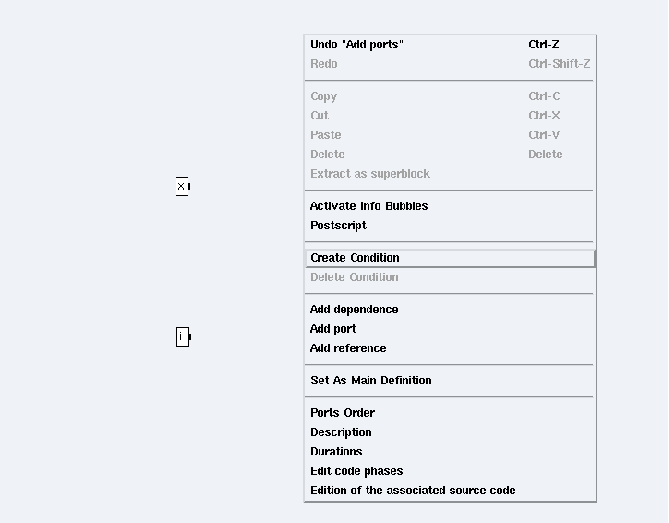Figure 5.1: Create Condition

• click on the condition x=1 (cf. figure 5.2) and create a reference div1<1> to the definition int/Arit_div,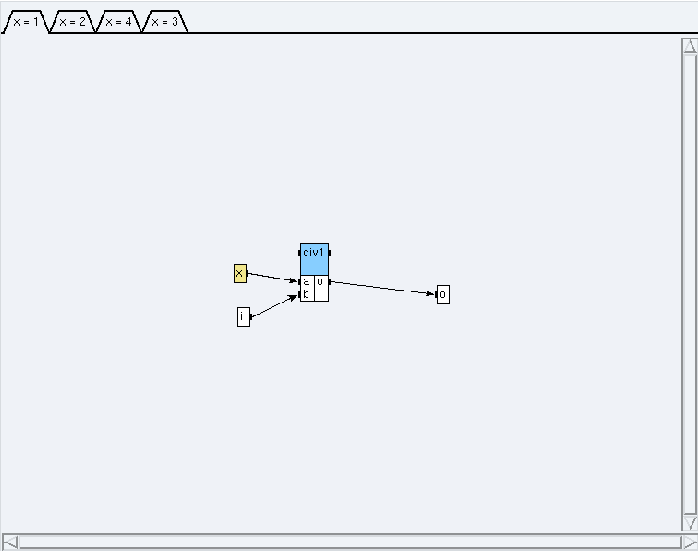Figure 5.2: Condition x=1

• click on the condition x=2 (cf. figure 5.3) and create a reference div2<1> to the definition int/Arit_div,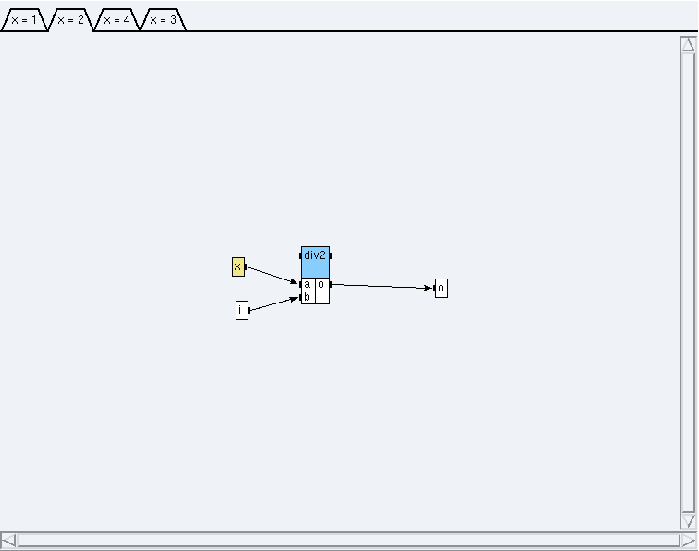Figure 5.3: Condition x=2

• click on the condition x=3 (cf. figure 5.4) and connect the port i to the port o,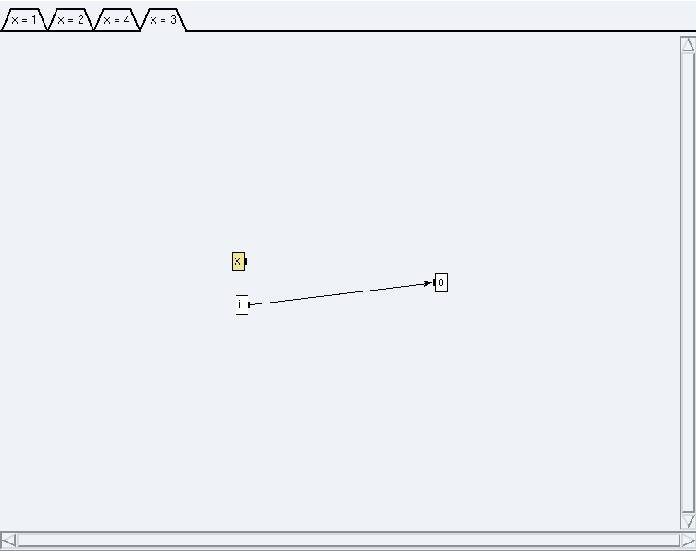Figure 5.4: Condition x=3

• click on the condition x=4 (cf. figure 5.5) and create a reference mul4<1> to the definition int/Arit_mul;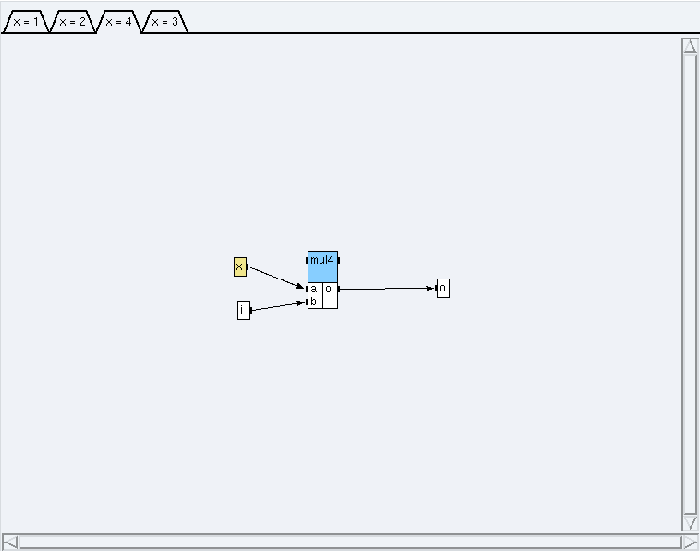Figure 5.5: Condition x=4

• create dependences between the references.

### 5.1.3  Algorithm AlgorithmMain1

The algorithm looks like the figure 5.6.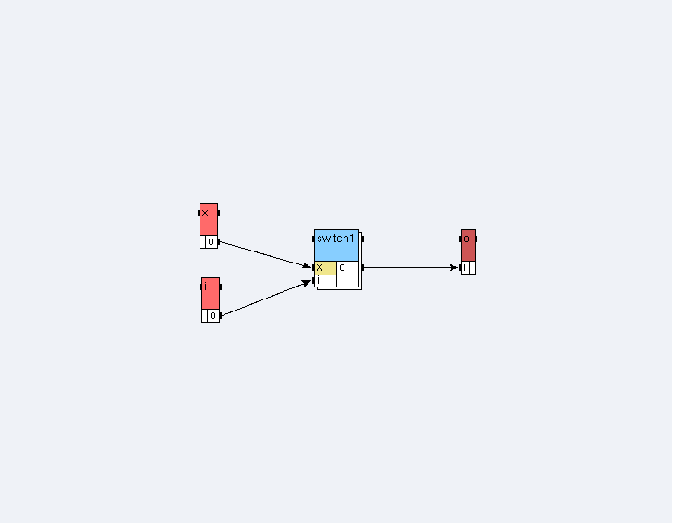Figure 5.6: AlgorithmMain1 of the Example 5

## 5.2  An algorithm with nested condition

### 5.2.1  Sensors x and i, actuator o

Use previous definitions (cf. 5.1.1).

### 5.2.2  Function switch2

To create the switch2 function:

• from the algorithm window: + green button → dialog window: switch2;
• in its definition window:
• contextual menu → Add port → dialog window: ? int x ? int y ! int o,
• contextual menu → Create Condition → dialog window: y=1 y=2,
• click on the condition y=1 (cf. figure 5.7) and create the function mul1<1> of the definition Arit_mul from int library,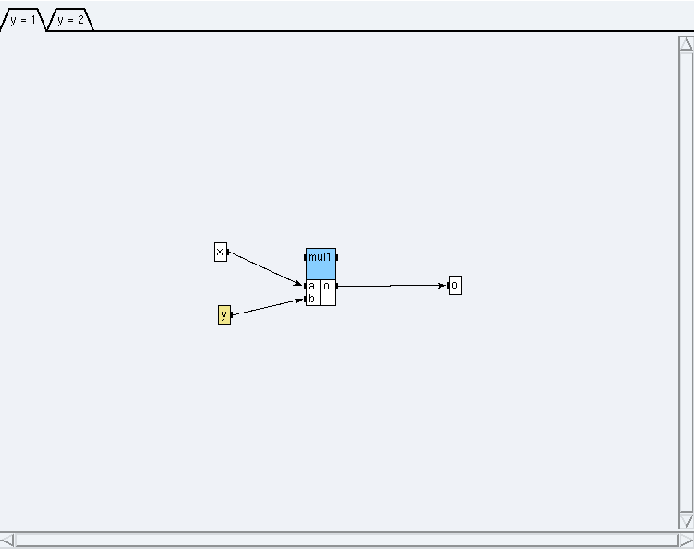Figure 5.7: Condition y=1

• click on the condition y=2 (cf. figure 5.8) and create a reference switch1 to the definition switch1;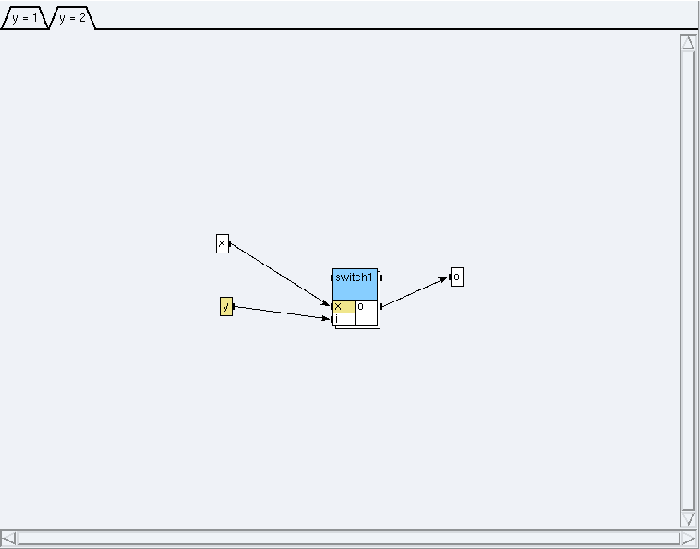Figure 5.8: Condition y=2

• create dependences between the references.

### 5.2.3  Algorithm AlgorithmMain2

The algorithm looks like the figure 5.9.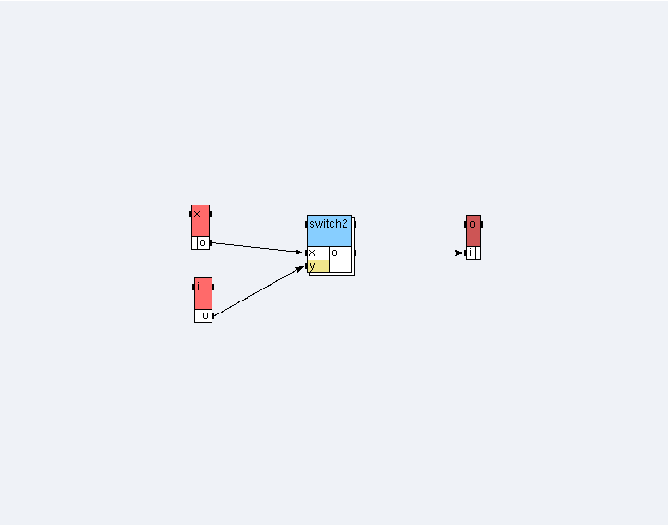Figure 5.9: AlgorithmMain2 of the Example 5

From the principal window, choose File / Close. In the dialog window, click on the Save button.

# Chapter 6  Example 6: algorithm, architecture, adequation, and code generation

From the principal window, choose File / Save as and save your sixth application under a new folder of your tutorial folder (e.g. my_example6) with the name example6.

## 6.1  The main algorithm

Create the main algorithm algo (cf. figure 6.1) using the library int for the operations In<1> (input), cste2<{2}> (cst), add<1> (Arit_add), mul<1> (Arit_mul), visuadd<1>, and visumul<1> (output). For the operation conv, create a function definition conv, create a reference to this definition, and give it a duration. Create the dependences between the references. Set it as main.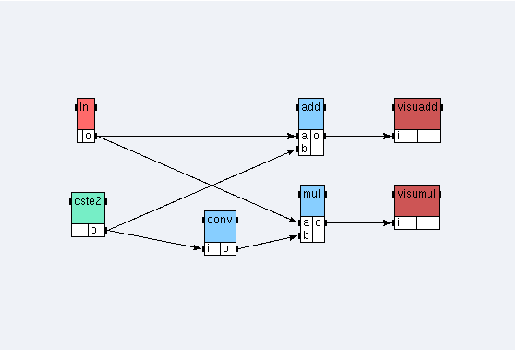Figure 6.1: Main algorithm of the Example 6

Warning: it is necessary that the sensor “in” should be distributed onto the processor “root”, i.e. on the local machine, in order that it operates properly.

## 6.2  The main architecture

To define and constraint the main architecture:

• include the library u,
• from the principal window, choose Architecture / Edit Architecture Definition and open the architecture biProc,
• define it as main,
• create the operation groups og1 and og2 then create the absolute constraints on the operators: og1 on root, og2 on P1. Attach references to operation groups: In, add, and visumulog1, mul, and visuaddog2.

## 6.3  The adequation and the code generation

To perform the adequation and to generate the code:

• before performing the code generation, you have to perform the adequation. Set the possibly missing durations, then from the principal window: Adequation / Launch Adequation;
• from the principal window: Code / Generate Executive(s). It generates for each operator of the main architecture the code in a file (files root.m4 and P1.m4) and an architecture description (file example6.m4). These files are generated in the same folder as the application. The files generated for each processor may be viewed: Code / Display Executive(s);
• the macros corresponding to the operations, included in the libraries of SynDEx, are already defined under the folder macros (files int.m4x, U.m4x, and TCP.m4x);
• in the same folder as the SynDEx application of the Example 6, create a new file example6.m4x in which you define the macro corresponding to the operation conv which is the only one not defined in the library, and the number of iterations. The file looks like:
```
dnl (c)INRIA 2001-2009
dnl SynDEx v7 executive macros specific to application tutorial/example6/example6
divert(-1)

define(‘NOTRACEDEF’)
define(‘NBITERATIONS’,3)
define(‘BINPWD’, ‘pwd’)
define(‘RSHELL’, ‘ssh’)

define(‘conv’,‘ifelse(
MGC,‘INIT’,‘dnl’,
MGC,‘LOOP’,‘\$2 = \$1 + 1;’,
MGC,‘END’,‘dnl’)’)

divert
divert(-1)
divert‘’dnl—————- end of file ——————

```
• create a new file example6.m4m, in which you set for each operator, except the main operator, the name of a workstation corresponding to this operator. The file looks like:
```
dnl (c)INRIA 2001-2009
define(‘P1_hostname_’, HOSTNAME)dnl

```
where HOSTNAME is the name of the remote workstation, as indicated in the Readme file under
syndex/examples/tutorial/example6;

Warning: the characters and are different.
Warning: if you increase the number of processors in your architecture, you must add other define statements with the corresponding “processor name”, “remote worksation name” association.

• create a new file root.m4x for the main operator including the file example6.m4m:
```
dnl (c)INRIA 2001-2009
include(example6.m4m)

```
• create a new file GNUmakefile which allows the compilation and the substitution of the macros from the code generation by the executable code. The file looks like:
```
# (c)INRIA 2001-2009
A  = example6
M4 = m4

export SynDEx_Path = SYNDEXPATH
export Algo_Macros_Path = \$(SynDEx_Path)/macros/algo_libraries
export Archi_Macros_Path = \$(SynDEx_Path)/macros/archi_libraries
export M4PATH = \$(Algo_Macros_Path):\$(Archi_Macros_Path)

CFLAGS = -DDEBUG
VPATH = \$(M4PATH)

.PHONY: all clean
all : \$(A).mk \$(A).run
clean ::
\$(RM) \$(A).mk

\$(A).mk : \$(A).m4 syndex.m4m U.m4m \$(A).m4m
\$(M4) \$< >\$@

root.libs =
P1.libs =

include \$(A).mk

```

where SYNDEXPATH is the absolute path of the SynDEx distribution.
Warning: in the previous makefile the statements \$(RM) \$(A).mk and \$(M4) \$< >\$@ must begin with a tab character.
The folder of the Example 6 must contain the following files:

• example6.m4
• example6.m4m
• example6.m4x
• example6.sdc
• example6.sdx
• GNUmakefile
• p1.m4
• root.m4
• root.m4x

To launch the execution, type the command make in the folder of the Example 6. To delete the file created during the compilation, type the command gmake clean.

From the principal window, choose File / Close. In the dialog window, click on the Save button.

# Chapter 7  Example 7: source code associated with an operation

## 7.1  Definition of the source code into the code editor window

In the previous Example 6, we have learnt that a m4 file, called with the name of the application plus the m4x extension, must be manually written. It contains all the source code associated with all operations present in a SynDEx application. For example, in the example6 application, the conv function increments of one the value of the input and stores the result in the output. Thus example6.m4x file contains:

```
define(‘conv’,‘ifelse(
MGC,‘INIT’,‘dnl’,
MGC,‘LOOP’,‘\$2 = \$1 + 1;’,
MGC,‘END’,‘dnl’)’)

```

where \$1 and \$2 correspond respectively to the input port named i and the output port named o of the conv function.

Handwriting this kind of code is not very easy, for several reasons:

• the port number may change by inserting or removing a port or parameter, following the SynDEx’s rule of port numeration. For example, after inserting a parameter P in the function conv, \$1 will not refer to the input port i but it will refer to the new added parameter P. All numbers are now brought, thus we must modify the code and replace the arguments \$1 by \$2 and \$2 by \$3;
• this task is quite repetitive when an application contains many operations;
• it is easy to make a mistake in the m4 syntax. Great knowledges in m4 syntax (two different kind of quotes, ifelse ...) and SynDEx m4 macros (MGC) are required.

It should be more convenient to write @OUT(o) = @IN(i) + @PARAM(P) and let SynDEx interpret it and generate the associated m4x file than to write the specification with the m4 syntax. SynDEx (version ≥ 7.0.0) is able to do that thanks the code editor which is a tool integrated in the graphical user interface (GUI). In the following example, we will show how to use this tool.

In this example, we show how to modify some functions by adding parameters in order to expand parameters into m4 arguments.

#### Open the example6.sdx application:

• from the principal window; choose File / Open → Open the example6.sdx file;
• save it as example7.sdx under a new folder of your tutorial folder (e.g. my_example7).

#### Add parameters to the conv function:

• open the conv definition and add parameter names P;T in the Parameters field,
• open the main algorithm definition, select the conv reference, add parameters values 2;3 in the Parameters field and modify the name reference from conv to conv_ref in the Name field.

#### Verify that the parameters have been stored in the function

We have two solutions:

• from the algorithm window on the main algorithm: put the mouse on the conv box → Read the printed informations in the principal window;
• or right click on the algorithm window background and select Activate Info Bubbles → Point the mouse cursor on the conv operation box.

### 7.1.2  To edit the code associated with an operation

#### In the case of a generic processor

• Open the code editor

We need to launch the code editor of the selected operation. Let us consider the case of the conv_ref function. We have to do the following operations:

• in the main algorithm: double left click on the conv_ref blue box. It opens the conv definition window. In its contextual menu, select Edition of the associated source code. It opens the code editor. It looks like a window with three push-buttons and an editable text area. Each push-button corresponds to one specific phase of three (init, loop, and end phases). When one of the three buttons is pressed, the text area shows the associated source code;
• in the conv definition window, right click on the background and select Edit code phases → Select init and endOK;
• in the code editor window: init phase → write in the text area (which is empty) the following C language code. This code is understood as a generic code:
```
printf("Init phase of function \$0 for default processor.\n");

```
• do the same thing for the loop phase 1:
```
@OUT(o)=@IN(i)*@PARAM(T)+@PARAM(P);
printf("Loop phase of function \$0 for default processor = %i.\n", @OUT(o));

```
• and for the end phase:
```
printf("End phase of function \$0 for default processor.\n");

```
• in the code editor window: Edit / Apply changes to all phases. It saves all buffers of all edited phases;
• in the code editor window: OK (it also saves all buffers).

Notice the following points:

• you can not launch the code editor from a main algorithm or a read-only operation (definition coming from libraries);
• you can write a code associated with a herarchical operation meanwhile it will not be present in the applicationName_sdc.m4x file (the background color of the text area of the code editor is grey);
• it is important to recall that the code is common to all the references of the same operator. Only the values of parameters are specific of each instance.
• Verify that code is common to all references

We create a new reference to the conv function:

• in the main algorithm: create a reference conv_ref_bis<8;9> to the definition conv;
• in the main algorithm: first remove the link between the conv_ref and mul boxes. Second, link the conv_ref output to the conv_ref_bis input. Third, link the conv_ref_bis output to the mul b input;
• in the algorithm window left click on the Auto-position button and write 120 in the two entry texts → OK,
• open the code editor of this new box: the code is the same. To show that values of parameters are specific to the reference (and not to the definition), we must generate the m4 code like shown in the previous example (Menu Adequation / Launch Adequation then Code / Generate Executive(s)) and look in P1.m4 (Menu Code / Display Executive(s)). The file contains
```
conv(2,3,_algo_cste2_P1_o,_algo_conv_ref_o)
conv(8,9,_algo_conv_ref_o,_algo_conv_ref_bis_o)

```

#### In the case of an architecture with heterogeneous processors

Sometimes it is interesting for an operation to have different source codes depending on the type of processor. For example, a given processor type X may only offer assembly language as a programming interface. In such case, we must be able to provide (for example) C code for processors that support it, and assembly language for the X processor type. To support heterogeneous architecture, the code editor associates code to a triplet (phase, processor, operation). A special processor type Default is provided for processors that have not been associated with dedicated code. Its use allows to share a code between different processor types.

• Include a new processor type

From the principal window, choose File / Included Libraries → Select c40

• Define an new architecture:
• from the principal window, choose Architecture / Define Architecture (cf. figure 1.14) → In the entry text, write archi2. → OK;
• in the archi2 window: create a reference root to the operator C40 and define it as the main operator.
• Replace the conv_ref_bis box by a reference to bar_ref:
• create a new function definition named bar with one input port called in and one output port called out;
• in the algo definition select all the references using the mouse and copy them (right click → Copy),
• create a new main algorithm named algo2;
• paste in the algo2 definition window;
• in the algo2 definition window: first delete the conv_ref_bis reference, then create a reference bar_ref box to the definition bar, finally create the missing dependences.
• Insert code for C40 processor type to the bar function:
• in the algo2 definition window: double left click on the bar_ref blue box. It opens the bar definition window. In its contextual menu, select Edition of the associated source code. It opens the code editor;
• in the bar definition window, right click on the background and select Edit code phases → Select init and endOK;
• in the code editor window: Type of Processor: Select C40;
• in the code editor window: click on the init phase button and write in the text area the following code:
```
/* Hi, I am \$0 function, in init phase for C40 processor */

```
• in the code editor window: click on the loop phase button and write in the text area the following code:
```
@OUT(out) = @IN(in);
/* Hi, I am \$0 function, in loop phase for C40 processor */

```
• in the code editor window: click on the end phase button and write in the text area the following code:
```
/* Bye, I am \$0 function, in end phase for C40 processor */

```
• Insert code for U processor type to the bar function:
• in the code editor window: Type of Processor: Select U;
• in the code editor window: click on the init phase button and write in the text area the following code:
```
/* Hi, I am \$0 function, in init phase for U processor */

```
• in the code editor window: click on the loop phase button and write in the text area the following code:
```
@OUT(out) = @IN(in) * 42;
/* Hi, I am \$0 function, in loop phase for U processor */

```
• in the code editor window: click on the end phase button and write in the text area the following code:
```
/* Bye, I am \$0 function, in end phase for U processor */

```
• Modify the durations

Add c40/C40 = 1 at the end of the Durations text area for each definition referenced in algo2

#### Learn the macros of the code editor

The code editor comes with a set of predefined macros that alleviate the user from knowing the black magic of m4 processing.

The more useful ones are names translation macros. These macros translate port and parameter names to their internal representation as m4 parameters. We have already encountered such macros in what we have just done: `@IN`, `@OUT` and `@INOUT` are port name translation macros, and `@PARAM` is the parameter name translation macro. As a rule of thumb, you should use `@PARAM(x)` when you want to refer to a parameter `x` and `@IN(i)` (resp. `@OUT(o)` / `@INOUT(io)`) when you refer to an input port `i` (resp. output port `o` / input-output port `io`).

The code editor recognizes three more macros: `@NAME`, `@QUOTE` and `@TEXT`. These advanced macros are not used in this tutorial and the reader is refered to SynDEx user manual to learn more about it.

### 7.1.3  To generate m4x files

Before performing the code generation we have to perform the adequation:

• from the principal window, choose Code: Select Generate m4x Files;
• from the principal window, choose Code / Generate Executive(s);
• from the principal window, choose Code / Display Executive(s).

Two cases are possible:

• the check box Generate m4x Files has not been checked. For each operator of the main architecture a processor_name.m4 file containing m4 macro-code is produced. As previously explained an architecture description file (named example7.m4) is also produced;
• the check box Generate m4x Files has been checked. Then, two new files (example7.m4x and example7_sdc.m4x) are generated in the same folder as the application.

These two files constitute the Applicative kernel:

• the MyApplication_sdc.m4x file contains all m4 macro code associated with operations used in the SynDEx application. Each time code generation is triggered, this file is overwritten;
• the MyApplication.m4x file is an user editable file whose goal is to allow the user to complete the applicative kernel if needed. At code generation time, if this file does not exist then SynDEx creates a generic file (including the MyApplication_sdc.m4x file plus some other features), otherwise the existing file is kept.

The example7_sdc.m4x file contains the following code:

```
divert(-1)
# (c)INRIA 2001-2009
divert(0)

define(‘example7_bar’,‘bar’)
define(‘bar’,‘ifelse(
processorType_,‘C40’,‘ifelse(
MGC,‘INIT’, “/* Hi, I am \$0 function, in init phase for C40 processor */”,
MGC,‘LOOP’,“\$2 = \$1; /* Hi, I am \$0 function, in loop phase for C40 processor */”,
MGC,‘END’, “/* Bye, I am \$0 function, in end phase for C40 processor */”)’)’)
processorType_,‘U’,‘ifelse(
MGC,‘INIT’,“/* Hi, I am \$0 function, in init phase for U processor */”,
MGC,‘LOOP’,“\$2 = \$1 * 42; /* Hi, I am \$0 function, in loop phase for U processor */”,
MGC,‘END’, “/* Bye, I am \$0 function, in end phase for U processor */”)’,

define(‘example7_conv’,‘conv’)
define(‘conv’,‘ifelse(
processorType_,processorType_,‘ifelse(
MGC,‘INIT’,“printf("Init phase of function \$0 for default processor.\n");”,
MGC,‘LOOP’,“\$5=\$3*\$2+\$1;
printf("Loop phase of function \$0 for default processor =%i.\n", \$4);”,
MGC,‘END’,“printf("End phase of function \$0 for default processor.\n");”)’)’)

```

If the example7.m4x file did not exist at code generation time then it will contain the following code:

```
divert(-1)
# (c)INRIA 2001-2009
divert(0)

define(‘dnldnl’,“// ”)
define(‘NOTRACEDEF’)
define(‘NBITERATIONS’,“5”)

include(‘example7_sdc.m4x’)

divert
#include <stdio.h> /* for printf */
divert(-1)
divert‘’dnl

```

Deeper insights about the m4 macro language can be found in SynDEx user manual and GNU M4 manual.

• See the difference between executable codes:
• create the example7.m4m file (cf. 6.3);
• create the root.m4x file (cf. 6.3);
• create the GNUmakefile (cf. 6.3), containing:
```
\$(A).mk : \$(A).m4 syndex.m4m U.m4m C40.m4m \$(A).m4m
\$(M4) \$< >\$@

```
• define algo2 as main algorithm;
• define archi as main architecture, perform the adequation, generate the code, and run the GNUmakefile compilation You obtain a root.c file containing U code only (no C40 code);
• define archi2 as main architecture, perform the adequation, generate the code, and compile again. This time, only C40 code is present in the root.c file.

Notice that:

• adequation modifies original files example7.sdx and example7.sdc;
• code generation produces these files: example7.m4, root.m4, pc1.m4 (if archi is defined as main), example7_sdc.m4x (if Generate m4x files is set) and example7.m4x (if Generate m4x files is set and the file did not exist before);
• compilation produces these files: example7.mk, root, root.c, and root.root.o, and (if archi is defined as main) pc1, pc1.c, and pc1.pc1.o.

From the principal window, choose File / Close. In the dialog window, click on the Save button.

1
Macros of the code editor as @IN, @OUT, @PARAM, … are explained in the User Manual.

# Chapter 8  Example 8: a complete realistic application from adequation to execution

From the principal window, choose File / Save as and save your eighth application under a new folder of yout tutorial folder (e.g. my_example8) with the name example8.

## 8.1  The aim of the example

In the seven previous examples we have learnt how to use SynDEx’s GUI to create architectures, algorithms, launch adequation, obtain executive files... Now, we have sufficient knowledge to perform a simple automatic control application that will be executed on a multiprocessor architecture.

First the application is described and the system is defined in Scicos (the block diagram editor of the Scilab software1). Second the corresponding SynDEx application is created (using the Example 1 to 3 of the tutorial). This needs the generation of some C code following the method discussed in Example 7. Finally, we compile the application to obtain executable for several processors as it has been shown in Examples 6 and 7. SynDEx generates the code necessary to the communication between the processors.

## 8.2  The model

We consider a system of two cars. The second car C2 follows the car C1 trying to maintain the distance l while the acceleration and the deceleration of C1. We call: x1(t) the position of the first car; x2(t) the position of the second car plus l; x_1(t) and x_2(t) the speeds of two cars. We denote k1 and k2 the inverse of the car masses. We call r(t) the reference speed chosen by the first driver. We suppose that we are able to observe the speed of the first car and the distance between the cars.

We have the following fourth order (four degrees of liberty) system:

 x1= k1 u1    x2= k2 u2    y1= x_1     y2= x1 − x2     (1)

We will decompose the system into mono-input mono-output system S1 (u1,y1) and S2 (u2,y2). Denoting by uppercase letter the Laplace transform of the variables, we have Y1=k1 U1/s and Y2=(k1 U1k2 U2)/s2 where U1 is seen as a perturbation that we want reject in the second system.

A first proportional feedback U11(RY1) will insure the first car to follow the reference speed. The second controller will be proportional derivative U22 Y23 s Y2 (in fact we will suppose in the following diagram that the derivative of y2 is also observed). The coefficient ρ1 is obtained by placing the pole of the first loop:

 Y1=ρ1k1R/(s+ρ1k1).

The coefficient ρ2 and ρ3 are obtained by placing the pole of the transfer from U1 to Y2 in the closed loop system which is given by:

 Y2=U1k1/(s2+k2ρ3s +k2ρ2).

## 8.3  The controllers

The purpose of the controller of the C1 car is to follow the reference in speed given the first driver. It stabilizes the C1 speed around its reference speed by using pole placement. For example, gains are respectively: (0, -5, 0, 0, -5). The controller of second car stabilizes the distance between the two cars. It stabilizes y2 around 0 by pole placement. For example, gains are respectively: (4, 4,-4,-4).

The controler of C2 knows these informations and sends them electronically to C1. This remark is available for C1.

### 8.3.1  Block diagrams of controllers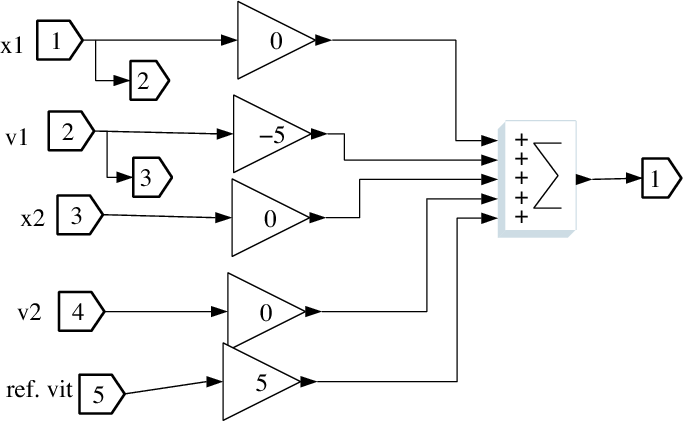Figure 8.1: Scicos controller of the first car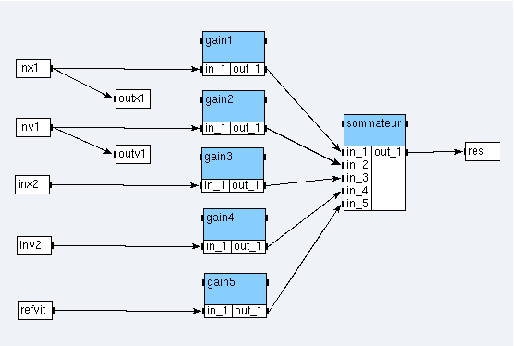Figure 8.2: SynDEx controller of the first car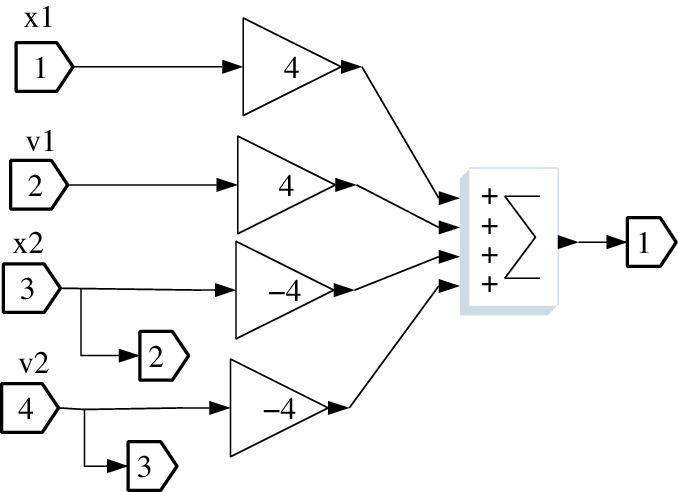Figure 8.3: Scicos controller of the second car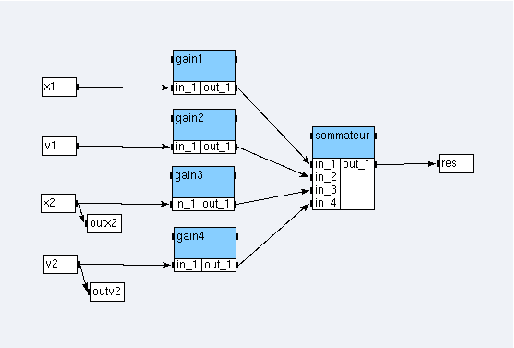Figure 8.4: SynDEx controller of the second car

Our controllers are simple. They are represented in figures 8.1 and 8.3 in Scicos and figures 8.2 and 8.4 in SynDEx:

• create a gain_def function with one input port in_1, one output port out_1 and a parameter named value. Notice that all ports will be of type float;
• then create sommateur2_def, sommateur4_def, and sommateur5_def functions, respectively with two input ports (in_1 and in_2), four input ports (from in_1 to in_4), and five input ports (from in_1 to in_5). Each of these three functions is created with only one output port out_1;
• finally create both algorithms controleur1_sup and controleur2_sup (cf. figures 8.2 and 8.4), setting their gain parameters respectively to 0, -5, 0, 0, 5 and 4, 4 ,-4 , -4.

### 8.3.2  Source code associated with the functions

We associate C source code to each function definition: gain and nary-sums. The code is inserted for the default processor.

#### Gain

A gain is a function that multiplies its input by a coefficient given as a parameter, named GAIN. After adding this parameter, open the code editor of the gain definition and write the following code in the loop phase of the default processor:

```
@OUT(out_1) = @IN(in_1) * @PARAM(GAIN);

```

#### Sum

We have three different forms of sum depending of its arity: two, four or five input ports:

• open the code editor of the sum function with two input ports and write the following code in the loop phase of the default processor:
```
@OUT(out_1) = @IN(in_1) + @IN(in_2);

```
• open the code editor of the sum function with the four input ports and write the following code in the loop phase of the default processor:
```
@OUT(out_1) = @IN(in_1) + @IN(in_2) +
@IN(in_3) + @IN(in_4);

```
• open the code editor of the sum function with the five input ports and write the following code in the loop phase of the default processor:
```
@OUT(out_1) = @IN(in_1) + @IN(in_2) +
@IN(in_3) + @IN(in_4) + @IN(in_5);

```

## 8.4  The complete model

In a real application, our job stops with the SynDEx’s adequation of the two controllers on their associated architectures. Nevertheless, for pedagogic reasons, we will simulate the whole system (with the dynamics of the cars) in the aim to verify that our application does the same job that Scicos.

### 8.4.1  The car dynamics

SynDEx is only used in discrete time model (not continuous time) and is not able to manage implicit algebraic loop. That is, in SynDEx, any loop contains at least a delay 1/z. Therefore, our application which is a continuous time dynamic system described in Scicos, must be discretized in time to be used in SynDEx.

The differential equation ẋ=u is discretized using the simplest way: the Euler scheme. Let us denote by h the step of the discretization and x0 an arbitrary initial value, the discretized system can be written as:

 xn+1 − xn= uh     (2)

Finally, the system is given in Scicos in the figure 8.5 and 2 is given in SynDEx in the figure 8.6. Notice that the variable h is stored in the Scicos context, and used in the input of the gain and the clock definition. In SynDEx, h is defined as parameter in the definition of a gain and the clock definition is directly used in the source code associated with operations.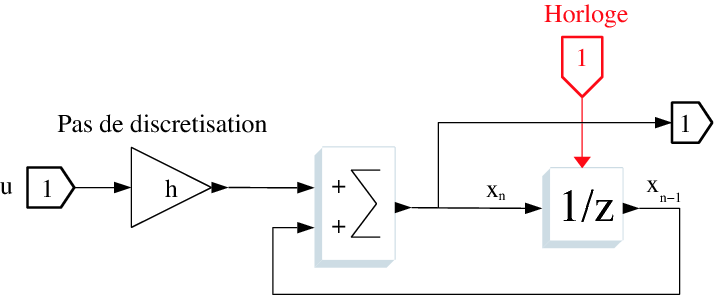Figure 8.5: An integral discretized in Scicos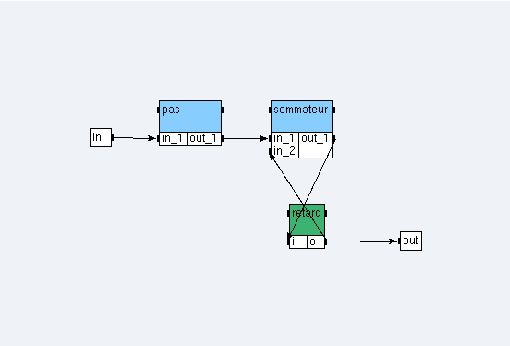Figure 8.6: An integral discretized in SynDEx

Create the integrale_discrete_sup algorithm (cf. figure 8.6). Notice that pas is of type gain_def with parameter GAIN equal to 0.001, sommateur is of type sommateur2_def, and retard is of type float/delay<{0};1>.

The car dynamics are given with Scicos block diagrams in the figure 8.7 and with SynDEx operations in the figure 8.8, where the input 1 (ref) is the acceleration of the car. The first integral gives the speed of the car and the second its position.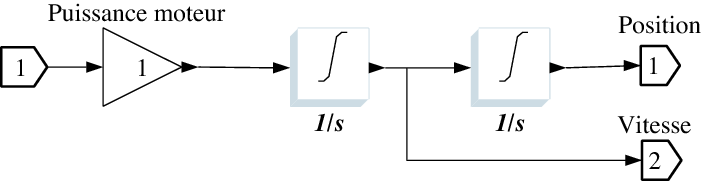Figure 8.7: Car dynamics with Scicos block diagrams (continous time)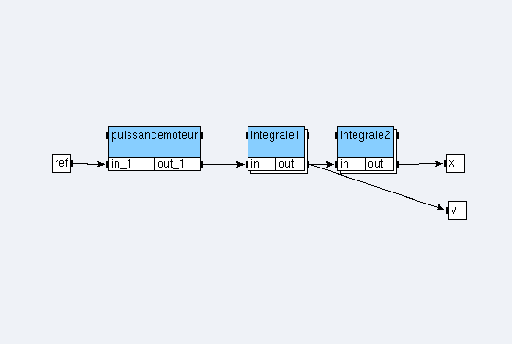Figure 8.8: Car dynamics with SynDEx operations

Create the mecanique_sup algorithm (cf. figure 8.8). Notice that puissancemoteur is of type gain_def with parameter GAIN equal to 1 whereas integrale1 and integrale2 are of type integrale_discrete_sup.

### 8.4.2  The cars and their controllers

In the following diagrams (from 8.9 to 8.12), the blocks (operations) denoted by meca are the car dynamics. Let us get the controllers of the two cars.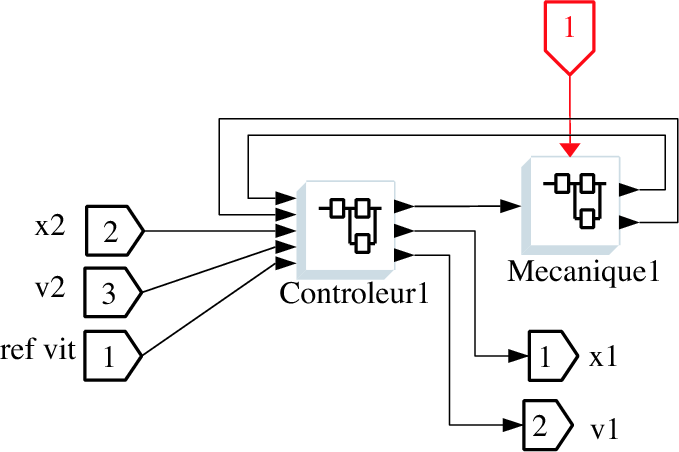Figure 8.9: Scicos C1 car dynamics and its controller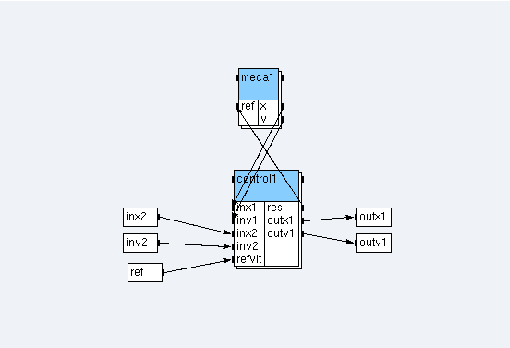Figure 8.10: SynDEx voiture1_sup car dynamics and its controller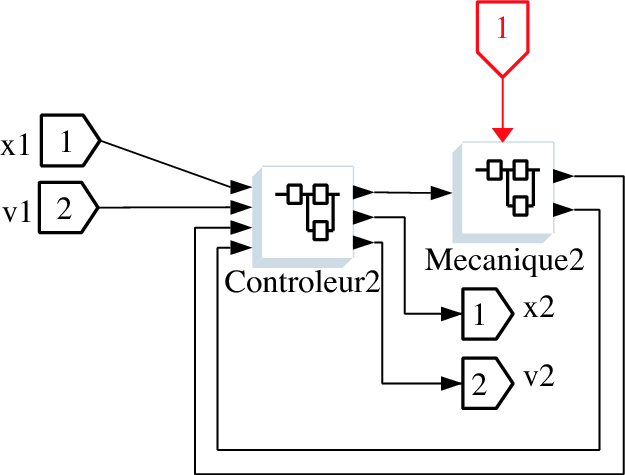Figure 8.11: Scicos C2 car dynamics and its controller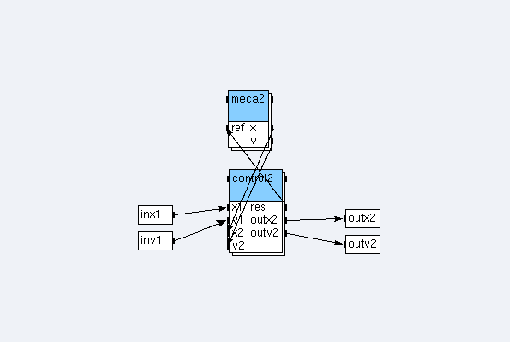Figure 8.12: SynDEx voiture2_sup car dynamics and its controller

Create the voiture1_sup and voiture2_sup algorithms (cf. figures 8.10 and 8.12). Notice that meca1 and meca2 are references to mecanique_sup whereas control1 (resp. control2) is a reference to controleur1_sup (resp. controleur2_sup).

### 8.4.3  The main algorithm

Create the following definitions (definitionName<PARAM>):

• senseur_def<POSI_ARRAY> with one output port out_1,
• vitesse_def<POSI_ARRAY> with one input port in_1,
• scope_def<POSI_ARRAY> with two input ports in_1 and in_2.

Then create algomain. Create the reference speed ref_vit of definition senseur_def<0>, the reference vitesse x_1 of definition vitesse_def<1>, the reference distance between the two cars of definition scope_def<2>, the reference vehicule1 of definition voiture1_sup and the reference vehicule2 of definition voiture2_sup, connect them according to the figure 8.13.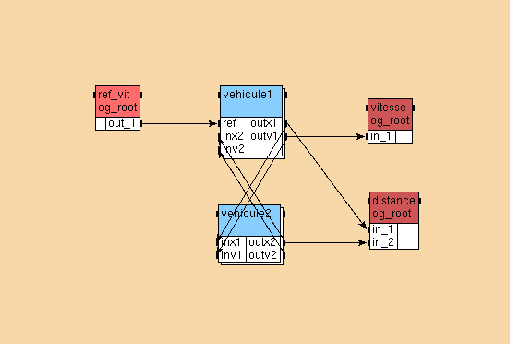Figure 8.13: Main algorithm

### 8.4.4  Source code associated with the sensor and the actuator

We associate C source code to each function definition: input and two kinds of output.

#### Input

In our Scicos application an input is a square wave generator. As a rule, we will simulate a square wave generator by reading values in a text file (named ref_vitesse.txt). We will use the fopen, the fclose and the fscanf functions (stdio.h library). We will also use assertions (assert.h library) to ensure that the opening of a file has been successful.

For the moment, let suppose that it exists an array of FILE* (the structure returned by the fopen function) called fd_array and a variable called timer to simulate a pseudo-timer. Our sensor has a parameter called POSI_ARRAY to remember the position of the FILE* structure in the array.

Now, open the code editor of the senseur_def sensor and write the following code in the init phase of the default processor:

```
timer = 0;
fd_array[@PARAM(POSI_ARRAY)] = fopen("ref_vitesse.txt", "r");
assert(fd_array[@PARAM(POSI_ARRAY)] != NULL);

```

In the Scicos application, we have defined the clock period of the square wave generator to the value 5 and the step of discretization h to the value 0.001. Thus we need, in the SynDEx application, to send 5000 times the same value. To count, we use the variable timer. All the 5000-th times, we read a new value in the file.

Write the following code in the loop phase of the default processor:

```
timer = (timer + 1) % 5000;
if (timer == 1)
fscanf(fd_array[@PARAM(POSI_ARRAY)], "%f\n", &data);
@OUT(out_1) = data;

```

We need to free memory by closing the file. Write the following code in the end phase of the default processor:

```
fclose(fd_array[@PARAM(POSI_ARRAY)]);

```

#### Speed output

An output saves in a file the values of the system states. Thus, an output has a parameter called POSI_ARRAY to remember the position of the array where the stream has been saved. Open the code editor of the vitesse_def actuator and write the following code in the init phase of the default processor:

```
fd_array[@PARAM(POSI_ARRAY)] = fopen("actuator_@TEXT(@PARAM(POSI_ARRAY))", "w");
assert(fd_array[@PARAM(POSI_ARRAY)] != NULL);

```

The loop phase, allows to save the values:

```
fprintf(fd_array[@PARAM(POSI_ARRAY)], "%E\n", @IN(in_1));

```

We need to free the memory by closing the file. Write the following code in the end phase:

```
fclose(fd_array[@PARAM(POSI_ARRAY)]);

```

#### Distance output

Contrary to the first type of output, this output has two input ports but the init and end source codes are identical. The loop phase differs. Open the code editor of the scope_def actuator and write the following code in the init phase of the default processor:

```
fprintf(fd_array[@PARAM(POSI_ARRAY)], "%E\n", (@IN(in_1) - @IN(in_2)));

```

### 8.4.5  The example8_sdc.m4x

SynDEx’s code generation will create the example8_sdc.m4x file (as explained in Example 7):

```
define(‘example8_algomain’,‘algomain’)
define(‘algomain’,‘ifelse(
processorType_,processorType_,‘ifelse(
MGC,‘INIT’,“”,
MGC,‘LOOP’,“WARNING: empty code for macro \$0 in loop phase”,
MGC,‘END’,“”)’)’)

define(‘example8_controleur1_sup’,‘controleur1_sup’)
define(‘controleur1_sup’,‘ifelse(
processorType_,processorType_,‘ifelse(
MGC,‘INIT’,“”,
MGC,‘LOOP’,“WARNING: empty code for macro \$0 in loop phase”,
MGC,‘END’,“”)’)’)

define(‘example8_controleur2_sup’,‘controleur2_sup’)
define(‘controleur2_sup’,‘ifelse(
processorType_,processorType_,‘ifelse(
MGC,‘INIT’,“”,
MGC,‘LOOP’,“WARNING: empty code for macro \$0 in loop phase”,
MGC,‘END’,“”)’)’)

define(‘example8_gain_def’,‘gain_def’)
define(‘gain_def’,‘ifelse(
processorType_,processorType_,‘ifelse(
MGC,‘INIT’,“”,
MGC,‘LOOP’,“WARNING: empty code for macro \$0 in loop phase”,
MGC,‘END’,“”)’)’)

define(‘example8_integrale_discrete_sup’,‘integrale_discrete_sup’)
define(‘integrale_discrete_sup’,‘ifelse(
processorType_,processorType_,‘ifelse(
MGC,‘INIT’,“”,
MGC,‘LOOP’,“WARNING: empty code for macro \$0 in loop phase”,
MGC,‘END’,“”)’)’)

define(‘example8_scope_def’,‘scope_def’)
define(‘scope_def’,‘ifelse(
processorType_,processorType_,‘ifelse(
MGC,‘INIT’,“”,
MGC,‘LOOP’,“WARNING: empty code for macro \$0 in loop phase”,
MGC,‘END’,“”)’)’)

define(‘example8_senseur_def’,‘senseur_def’)
define(‘senseur_def’,‘ifelse(
processorType_,processorType_,‘ifelse(
MGC,‘INIT’,“”,
MGC,‘LOOP’,“WARNING: empty code for macro \$0 in loop phase”,
MGC,‘END’,“”)’)’)

define(‘example8_sommateur2_def’,‘sommateur2_def’)
define(‘sommateur2_def’,‘ifelse(
processorType_,processorType_,‘ifelse(
MGC,‘INIT’,“”,
MGC,‘LOOP’,“WARNING: empty code for macro \$0 in loop phase”,
MGC,‘END’,“”)’)’)

define(‘example8_sommateur4_def’,‘sommateur4_def’)
define(‘sommateur4_def’,‘ifelse(
processorType_,processorType_,‘ifelse(
MGC,‘INIT’,“”,
MGC,‘LOOP’,“WARNING: empty code for macro \$0 in loop phase”,
MGC,‘END’,“”)’)’)

define(‘example8_sommateur5_def’,‘sommateur5_def’)
define(‘sommateur5_def’,‘ifelse(
processorType_,processorType_,‘ifelse(
MGC,‘INIT’,“”,
MGC,‘LOOP’,“WARNING: empty code for macro \$0 in loop phase”,
MGC,‘END’,“”)’)’)

define(‘example8_vitesse_def’,‘vitesse_def’)
define(‘vitesse_def’,‘ifelse(
processorType_,processorType_,‘ifelse(
MGC,‘INIT’,“”,
MGC,‘LOOP’,“WARNING: empty code for macro \$0 in loop phase”,
MGC,‘END’,“”)’)’)

define(‘example8_voiture1_sup’,‘voiture1_sup’)
define(‘voiture1_sup’,‘ifelse(
processorType_,processorType_,‘ifelse(
MGC,‘INIT’,“”,
MGC,‘LOOP’,“WARNING: empty code for macro \$0 in loop phase”,
MGC,‘END’,“”)’)’)

define(‘example8_voiture2_sup’,‘voiture2_sup’)
define(‘voiture2_sup’,‘ifelse(
processorType_,processorType_,‘ifelse(
MGC,‘INIT’,“”,
MGC,‘LOOP’,“WARNING: empty code for macro \$0 in loop phase”,
MGC,‘END’,“”)’)’)

```

### 8.4.6  To handwrite the example8.m4x file

You will not can use directly the SynDEx’s generated example8.m4x generic file because both the creation of local variable and the call of libraries is missing. After the code generation, you will must handwrite it to obtain the following code:

```
define(‘dnldnl’,“// ”)
define(‘NOTRACEDEF’)
define(‘NBITERATIONS’,“20000”)

define(‘BINPWD’, ‘pwd’)
define(‘RSHELL’, ‘ssh’)

define(‘proc_init_’,‘
FILE *fd_array;
float data;
int timer;’)

include(‘example8_sdc.m4x’)

divert
divert(-1)
divert‘’dnl

```

Where the macro proc_init_ allows the local variable declaration to be declared because it inserts its source code between the main function and the operations initialization. Notice that the main loop of the program is defined generically with a loop of NBITERATIONS where NBITERATIONS is initialized with the size of the input file (ref_vitesse.txt). Finally, the call of libraries is inserted after the include of the example8_sdc.m4x file.

## 8.5  Scicos simulation

Scicos software allows to simulate models in a window (cf. figure 8.14), where the values of three states are plotted (ordinate axle) according to the time (abscissa axle). We have:

• the square wave generator drawn at the bottom (red);
• the speed of the first car at the top (black);
• the distance between the two cars seen in the middle of the figure (green).

Thanks the diagram, the system is stable (plots do not grow exponentially) and so it works. We do not continue to ameliorate the controllers job.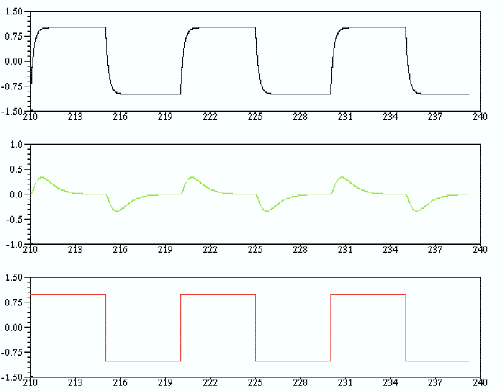Figure 8.14: Scope window obtained with the values 0; -5; 0; 0; -5 for gains of the C1 controller and 4; 4; -4; -4 for the C2 controller.

## 8.6  SynDEx simulation

### 8.6.1  In the case of a mono-processor architecture

#### The architecture

In this subsection, we suppose that the architecture is constituted of an only operator named root:

• create the U operator:
• set the durations with
```
float/delay = 1
gain_def = 1
scope_def = 1
senseur_def = 2
sommateur2_def = 2
sommateur4_def = 1
sommateur5_def = 2
vitesse_def = 1

```
• choose init as code generation phase in which to generate code;
• create the mono architecture:
• add one U operator named root and define it as main operator,
• define the mono architecture as main.

#### The adequation and the code generation

First, launch the adequation. It modifies example8.sdc and example8.sdx files.

Then, generate the executive and applicative files (setting Code / Generate m4x Files). It creates example8.m4, example8.m4x, example8_sdc.m4x, and root.m4 files.

Finally, handwrite the example8.m4x file as explained in 8.4.6.

#### The compilation

First, generate manually a GNUmakefile containing:

```
A  = example8
M4 = gm4

export ArchiMacros_Path = ../../../macros/archi_libraries
export AlgoMacros_Path = ../../../macros/algo_libraries
export M4PATH = \$(ArchiMacros_Path):\$(AlgoMacros_Path)

CFLAGS = -DDEBUG
VPATH = \$(M4PATH)

.PHONY: all clean
all : \$(A).mk \$(A).run
clean ::
\$(RM) \$(A).mk *~ *.o *.a *.c actuator_*

\$(A).mk : \$(A).m4 syndex.m4m U.m4m
\$(M4) \$< >\$@

root.libs =
P1.libs =

include \$(A).mk

```

Where:

• A is set with the name of your application (here example8);
• the path about the generic *.m4? macro-files are stored in the exported shell variables ArchiMacros_Path and AlgoMacros_Path then grouped into a new exported shell variable named M4PATH. The separator : means that a m4 macro-file will be search first in ArchiMacros_Path and then in AlgoMacros_Path if is not found;
• a mix of this makefile and the informations stored in file named example8.m4m will create another makefile called example8.mk during the compilation.

Then, copy-paste the ref_vitesse.txt file from the Example 8 folder to yours.

Then, type the command gmake in a shell commands interpreter. It creates actuator_1, actuator_2, example8.mk, root, root.c, and root.root.o files:

• the actuator_1 file contains the speed of the first car;
• the actuator_2 file contains the distance between the cars.

### 8.6.2  In the case of a bi-processor architecture

#### The architecture

In this subsection, we suppose that the architecture is constituted of two operators named root and pc1, of type U and linked with a medium tcp1 of type TCP:

• create the TCP medium:
• set the type with SAM MultiPoint,
• set the durations with float = 1;
• modify the U operator: set the gates with TCP x and TCP y;
• create the biProc architecture:
• add one U operator named root and define it as main operator,
• add one U operator named pc1,
• add one TCP medium named tcp1 and choose the Broadcast option,
• links the medium to the x gates of the operators,
• define the biProc architecture as main.

#### The adequation and the code generation

First, launch the adequation. It modifies example8.sdc and example8.sdx files.

Then, generate the executive and applicative files (setting Code / Generate m4x Files). It creates example8.m4, example8.m4x, example8_sdc.m4x, root.m4, and pc1.m4 files.

Finally, handwrite the example8.m4x file as explained in 8.4.6.

#### The compilation

First, generate manually the GNUmakefile, the example8.m4m, and the root.m4x files:

• the GNUmakefile has to be created as explained in 8.6.1. Upgrade it so that the line:
```
\$(A).mk : \$(A).m4 syndex.m4m U.m4m

```
is changed by:
```
\$(A).mk : \$(A).m4 syndex.m4m U.m4m \$(A).m4m

```
• create the example8.m4m file with this line: define(‘pc1_hostname_’, HOSTNAME)dnl where HOSTNAME is substituted with the name of your remote station;
• create the root.m4x file with this line: include(example8.m4m).

Then, copy-paste the ref_vitesse.txt file from the Example 8 folder to yours.

Then, type the command gmake in a shell commands interpreter. It creates actuator_1, actuator_2, example8.mk, root, root.c, root.root.o, pc1, pc1.c, and pc1.pc1.o files:

• the actuator_1 file contains the speed of the first car;
• the actuator_2 file contains the distance between the cars.

### 8.6.3  In the case of a multi-processor architecture

#### The architecture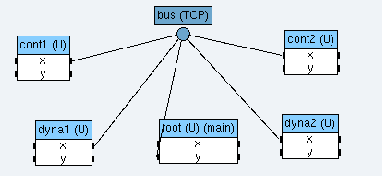Figure 8.15: The architecture with 5 operators.

In this subsection, we suppose that the architecture is constituted of five operators named root,cont1, cont2, dyna1, and dyna2, of type U and linked with a medium bus of type TCP:

• create the multi architecture (cf. figure 8.15):
• add one U operator named root and define it as main operator,
• add one U operator named cont1,
• add one U operator named cont2,
• add one U operator named dyna1,
• add one U operator named dyna2,
• add one TCP medium named bus and choose the Broadcast option,
• links the medium to the x gates of the operators, except for the root one, linked with its y gate to the medium,
• define the multi architecture as main;
• create operation groups:
• create the og_root operation group, attach ref_vit, vitesse, and distance to it,
• create the og_dyna1 operation group, from the main mode, in the vehicule1 reference attach meca1 to it,
• create the og_cont1 operation group, from the main mode, in the vehicule1 reference attach control1 to it,
• create the og_dyna2 operation group, from the main mode in the vehicule2 reference attach meca2 to it,
• create the og_cont2 operation group, from the main mode, in the vehicule2 reference attach control2 to it;
• create absolute constraints:
• constrain og_root on root,
• constrain og_cont1 on cont1,
• constrain og_cont2 on cont2,
• constrain og_dyna1 on dyna1,
• constrain og_dyna2 on dyna2.

#### The adequation and the code generation

First, launch the adequation. It modifies example8.sdc and example8.sdx files.

Then, generate the executive and applicative files (setting Code / Generate m4x Files). It creates example8.m4, example8.m4x, example8_sdc.m4x, root.m4, cont1.m4, cont2.m4, dyna1.m4, and dyna2.m4 files.

Finally, handwrite the example8.m4x file as explained in 8.4.6.

#### The compilation

First, generate manually the Makefile.ocaml, the example8.m4m, the root.m4x, the cont1.m4x, the cont2.m4x, the dyna1.m4x, and the dyna2.m4x files:

• copy-paste the Makefile.ocaml from the Example 8 folder to yours;
• create the example8.m4m file with these lines:
```
define(‘cont1_hostname_’, HOSTNAME)dnl
define(‘cont2_hostname_’, HOSTNAME)dnl
define(‘dyna1_hostname_’, HOSTNAME)dnl
define(‘dyna2_hostname_’, HOSTNAME)dnl

```
where HOSTNAME is substituted with the name of your remote station;
• create the root.m4x, cont1.m4x, cont2.m4x, dyna1.m4x, and dyna2.m4x files with this line: include(example8.m4m).

Then, copy-paste some files from the Example 8 folder to yours:

• copy-paste the example8.ml file.
• copy-paste the pa_example8.ml file.
• copy-paste the root.sh, cont1.sh, cont2.sh, dyna1.sh, and dyna2.sh files;
• copy-paste the ref_vitesse.txt file.

You will probably need to install camlp5 (see at http://pauillac.inria.fr/ ddr/camlp5/).

Then, type the command make -f Makefile.ocaml in a shell commands interpreter. It creates example8.cmi, example8.o, pa_example8.cmi, and <processor>.cmi, <processor>.cmx, <processor>.o, <processor>.opt for each processor.

Finally, launch separatly the five script files. At the end of their execution, the actuator_1 and actuator_2 files are created:

• the actuator_1 file contains the speed of the first car;
• the actuator_2 file contains the distance between the cars.

From the principal window, choose File / Close. In the dialog window, click on the Save button.

1
http://www.scilab.org

# Chapter 9  Example 9: a multiperiodic application

From the principal window, choose File / Save as and save your ninth application under a new folder of yout tutorial folder (e.g. my_example9) with the name example9.

## 9.1  The main algorithm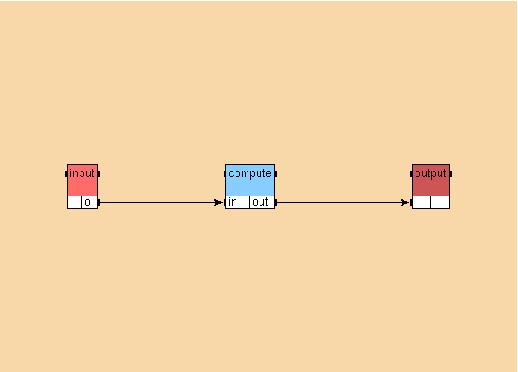Figure 9.1: Main algorithm of the Example 9

Create the main algorithm basicAlgorithm (cf. figure 9.1) using the library int for the operations input<1> (int/input) and output<1> (int/output). For the operation compute, create a function definition compute and create a reference to this definition. Create the dependences between the references. Set the periods to 4 for input, 8 for compute, and 8 for output by selecting each reference and filling the Period field.

## 9.2  The main architecture

Open the architecture monoProc from the library u. Define it as main. The durations for the U operator are by default:

```
int/input = 3
Implode_int = 1
compute = 1
int/output = 3

```

Implode_int is an internal operation automatically generated by SynDEx to collect the different data produced by the different occurences of the int/input operation.
In this case, the system is not schedulable.

## 9.3  A mono-phase schedule

### 9.3.1  Durations

Modify the durations for the U operator:

```
int/input = 1
Implode_int = 1
compute = 1
int/output = 1

```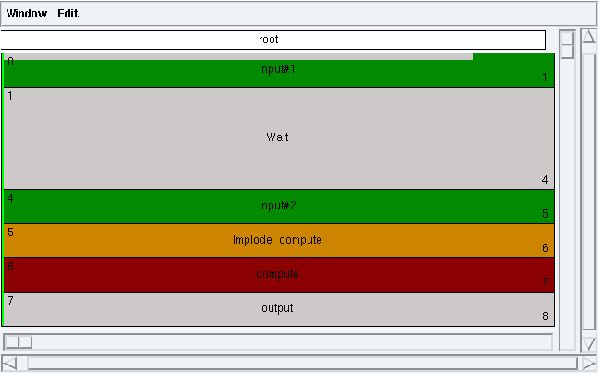Figure 9.2: A mono-phase schedule

#### Wait operation

Notice the new operation added by SynDEx (Wait) to respect the period of the input operations.

#### Multiple occurrences

Notice that because of the periods, during a cycle two input operations are executed (input#1 and input#2) whereas only one compute and one output operations are executed.

#### Implode operation

Notice the new operation added by SynDEx (Implode_compute) to provide the data from the input operations to the compute one.

## 9.4  A multi-phase schedule

### 9.4.1  Durations

Modify the durations for the U operator:

```
int/input = 1
Implode_int = 1
compute = 2
int/output = 1

```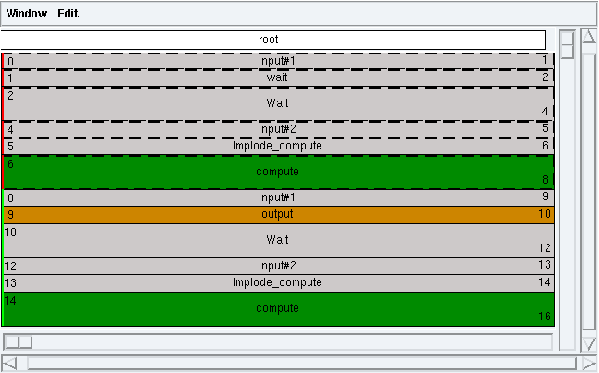Figure 9.3: A multi-phase schedule

Launch the adequation (Adequation / Launch Adequation). Display the schedule (Adequation / Display Schedule). The computed schedule has two phases: a transitory phase (red) and a permanent phase (green) (cf. figure 9.3).

#### Transitory phase

The transitory phase is executed only once. It contains the first occurrence of the input#1 operation, the first occurrence of the input#2 operation, the first occurrence of the Implode_compute operation, and the first occurrence of the compute operation. The compute operation provide data consumed by the output operation schedule at time 9 in the permanent phase.

#### Permanent phase

The permanent phase is the one that is executed infinitely. It contains the second occurrence of the input#1 operation (and its following occurrences). It contains the first occurrence of the output operation (and its following occurrences).

This document was translated from LATEX by HEVEA.